Do you like the article?
Share it with others —
Use new possibilities of MetaTrader 5

#### Similar articles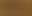# Elastic net regression using coordinate descent in MQL5

MetaTrader 5Examples | 12 September 2023, 11:021 4220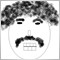### Introduction

Elastic net regression combines the best qualities of the ridge and  lasso techniques to build general linear models. Applying it makes  it possible to minimize one of the main disadvantages of  regression, overfitting. This is especially relevant to trading  strategy development, as it is known, that the most common reason  for poor performance or strategy failure.Comes as a result of  mistaking noise for patterns during training. In this article we  will present the implementation of elastic net regression, that  uses the coordinate descent method of optimization in pure MQL5.  Towards the end of the article we will demonstrate a practical  application of this technique through the development of a simple  moving average based predictive strategy.

### Regularization

When building predictive models the goal is to create an exemplar  that can discern some unique pattern that can be exploited for real  world use. To do so effectively we have to ensure that the model is  "learning" relevant patterns from the training data. This is  obviously easier said than done. What usually happens is that the  model ends up picking up on irrelevant information (noise) which  ultimately hurts its performance when put to use. Regularization is  a process used to minimize the effects of overfitting.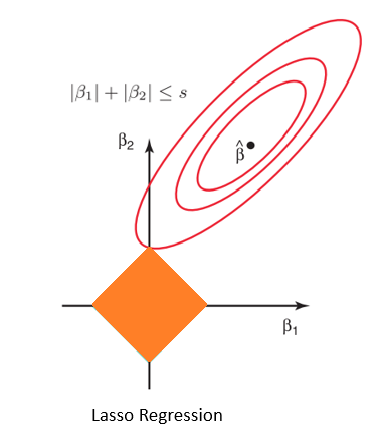The lasso technique helps reduce training bias by repressing  redundant predictors when the model is defined by too many  optimizable variables. Thereby simplifying the  model.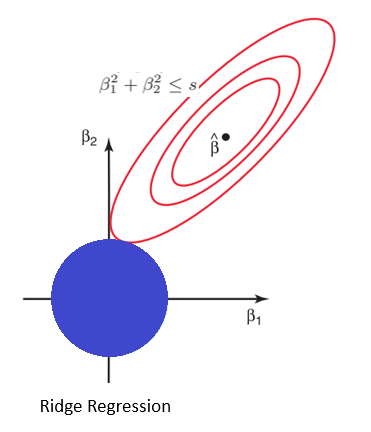In ridge  regression, coefficients of the regression equation are minimized  so as to move them away from their optimum. This helps to  generalize the model whilst still keeping all the predictors.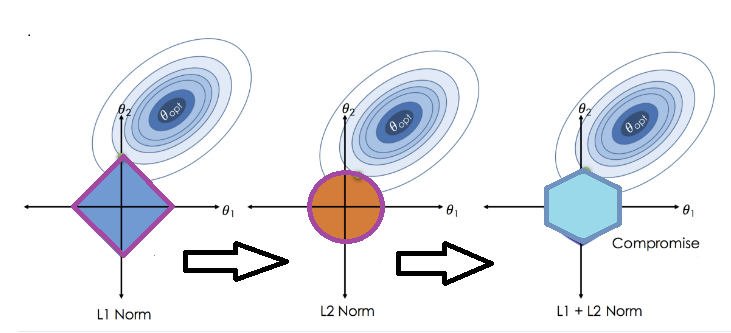Both the lasso and the ridge are distinguished by the nature of  the penalty term applied. The elastic net regression penalty term  is a combination of the absolute value and square of the  coefficents, weighted by two hyperparameters, alpha and lambda.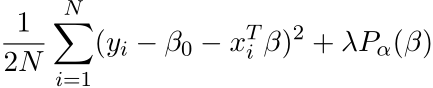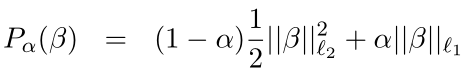This way, alpha controls the type of regularization. When alpha is  zero the penalty term reduces to the l2-norm, alternatively, when  alpha is 1 the penalty function becomes the l1-norm. Specification  of alpha that is between 0 and 1 makes it possible to build linear  models that combine the qualities of both ridge and lasso  regression to a certain degree as governed by the lambda  hyperparameter which controls the degree of regularization.

Such models can be a boon when applied to trading strategy  development where we often find ourselves blindly applying numerous  predictors in the hope of finding some combination that produces   profit. Using elastic net regression we can minimize overfitting  whilst also being able to separate useless indicators from those  that have significant predictive potential. And we can do this  without worrying about how the indicators are related to each  other. It almost seems too good to be true.

### Coordinate Descent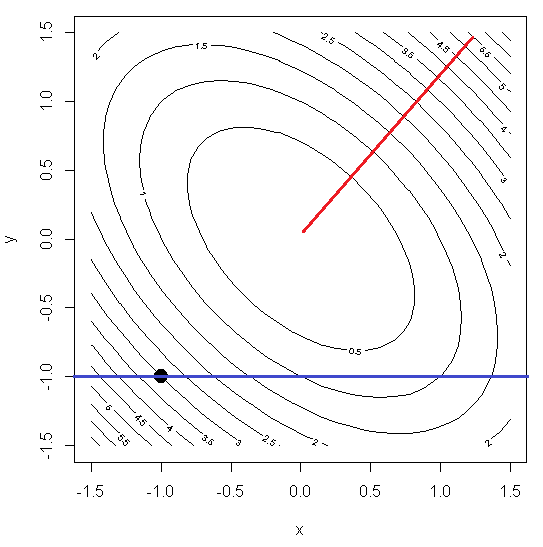Coordinate descent is a method of optimization well suited to multivariable optimization. A complex multidimensional optimization problem is reduced into a collection of one dimensional problems.  Achieved by iteratively minimizing each of the individual dimensions of a function , whilst keeping the values of the  function in other dimensions static. There are numerous resources  on the internet that can provide more detailed explanations for  those interested. Here, we are interested in its application to  strategy development.

For our purposes, the coordinate descent method will be used in two  ways in the implementation of elastic net regression. First it will  be used to determine the optimal lambda based on a fixed alpha,  specified by the user. Once this is done, the optimization method is  called again to work on the beta coefficients of the regression equation. Lets dive into some code to see how this is accomplished.

### The CCoordinateDescent class

```//+------------------------------------------------------------------+
//| Coordinate Descent optimization class                            |
//+------------------------------------------------------------------+
class CCoordinateDescent
{

private:
bool              m_initialized;   // Was everything legal and allocs successful?
double            m_beta[];        // Beta coefs (m_nvars of them)
double            m_explained;     // Fraction of variance m_explained by model; computed by Train()
double            m_xmeans[];      // Mean of each X predictor
double            m_xscales[];     // And standard deviation
double            m_ymean;         // Intercept (mean of Y)
double            m_yscale;        // Standard deviation of Y

int               m_nvars ;        // Number of variables
int               m_observs ;      // Number of cases
bool              m_covarupdates ; // Does user want (often faster) covariance update method?
int               m_nlambda ;      // Reserve space for this many m_beta sets for saving by TrainLambda() (may be zero)
double            m_lambdabeta_matrix[];  // Saved m_beta coefs (m_nlambda sets of m_nvars of them)
double            m_lambdas[];     // Lambdas tested by TrainLambda()
double            m_x_matrix[];           // Normalized (mean=0, std=1) X; m_observs by m_nvars
double            m_y[];           // Normalized (mean=0, std=1) Y
double            m_resid[];       // Residual
double            m_xinner_matrix[];      // Nvars square inner product matrix if m_covarupdates
double            m_yinner[];      // Nvars XY inner product vector if m_covarupdates

public:
//constructor
CCoordinateDescent(const int num_predictors, const int num_observations, const bool use_covariance_updates, const int num_lambdas_to_trial) ;
//desctructor
~CCoordinateDescent(void) ;

//Accessor methods for private properties
double            GetYmean(void)                     { return m_ymean; }
double            GetYscale(void)                    { return m_yscale;}
double            GetExplainedVariance(void)         { return m_explained;}

double            GetXmeansAt(const int index)       { if(index>=0 && index<ArraySize(m_xmeans)) return m_xmeans[index]; else return 0;}
double            GetXscalesAt(const int index)      { if(index>=0 && index<ArraySize(m_xscales)) return m_xscales[index]; else return 0;}
double            GetBetaAt(const int index)         { if(index>=0 && index<ArraySize(m_beta)) return m_beta[index]; else return 0;}
double            GetLambdaAt(const int index)       { if(index>=0 && index<ArraySize(m_lambdas)) return m_lambdas[index]; else return 0;}
double            GetLambdaBetaAt(const int index)   { if(index>=0 && index<ArraySize(m_lambdabeta_matrix)) return m_lambdabeta_matrix[index]; else return 0;}
double            GetLambdaThreshold(const double alpha) ;
//Set model parameters and raw input data
bool              SetData(const int begin, const int num_observations, double &xx_matrix[], double &yy[]) ;
//Training routines
void              Train(const double alpha, const double lambda, const int maxits, const double convergence_criterion, const bool fast_test, const bool warm_start) ;
void              TrainLambda(const double alpha, const int maxits, const double convergence_criterion, const bool fast_test, const double maxlambda, const bool print_steps) ;
} ;```

The CCoordinateDescent class is defined in the  CoordinateDescent.mqh file. Its constructor is parametric and is  used to specify important features of a model, but before we get  into this, there are some pertinent issues about a specific data construct to be used.

The library we will specify will not use any unique data types,  such as the new matrix and vector types of MQL5. This is to ensure  compatibility with mql4. Since there is no way to dynamically  define multidimensional arrays, matrices will be represented as  regular flat arrays. An example is the best way to illustrate the  construct.

Supose we want to define a matrix with 4 rows and 3 columns. We  would create an array of size 4 multiplied by 3 , ie 12. The  members of this array will be arranged as they would appear if  using the built in matrix data type. That is to say, using our  example, the first row members are specified first, then the second  row members, and so on. The code snippet below illustrates the creation of a 4 by 3 matrix , where the each value in a column is the same.

```int rows=4,
columns=3;

double our_matrix[];

ArrayResize(our_matrix,rows*columns);

/*
Creating matrix with columns of 1s,2s,3s
*/

for(int i = 0; i<rows; i++)
for(int j=0; j<columns; j++)
our_matrix[i*columns+j]=j+1;

ArrayPrint(our_matrix);
```

Output from ArrayPrint.

```KP 0  13:01:32.445   Construct(GBPUSD,D1)   1.00000 2.00000 3.00000 1.00000 2.00000 3.00000 1.00000 2.00000 3.00000 1.00000 2.00000 3.00000
```

When traversing the array in matrix fashion, we have the row index  * the number of columns + the column index. Instances where such array constructs are required are all denoted by the _matrix suffix to  either a class variable name or a function parameter name.

Using this construct means that when matices are passed to  functions, a few function parameters have to be reserved for the  specification of the dimensions of a particular matrix. I am sure  it will become a lot more intuitive once we get to  the application  of the class towards the end of the article. Users are free to fork  the library if not interested in cross platform portability. Back  to the description of the class.

```//+------------------------------------------------------------------+
//|Constructor                                                       |
//+------------------------------------------------------------------+
CCoordinateDescent::CCoordinateDescent(
const int  num_predictors,   // Number of predictor variables
const int num_observations,    // Number of cases we will be training
const bool use_covariance_updates,    // Use fast covariance updates rather than slow naive method
const int num_lambdas_to_trial     // Number of m_lambdas we will be using in training
)```

The parametric constructor requires 4 parameters:

• num_predictors sets the number of variables, this is the number of  predictors or indicators, each set of indicators will occupy a  column in the internal data matrix.
• num_observations specifies the amount of data the object should  expect, this would be the number of rows or the exact number  elements available in each set of variables/predictors/indicators.
• use_covariance_updates is a boolean option that should ideally be  used when there are more more num_observations than  num_predictors.Setting it to true provides significant improvements  in excecution time relative to the alternative. This option should  be considered only when num_observations > num_predictors.
• num_lambdas_to_trial sets the maximum number of lambda variations  that will be tested during the training process.

The constructor simply prepares the internal data structures to  receive all the required data.

``` {
m_nvars = num_predictors ;
m_observs = num_observations ;
m_nlambda = num_lambdas_to_trial ;
m_initialized=true;
m_ymean=m_yscale=m_explained=0;

if(m_nvars<0 || m_observs<0 || m_nlambda<0)
{
m_initialized=false;
Print("Invalid parameter value, neither num_predictors ,num_observations, nor num_lambdas_to_trial can be negative");
return;
}

if(ArrayResize(m_x_matrix,m_observs*m_nvars)<m_observs*m_nvars    ||
ArrayResize(m_y,m_observs)<m_observs                ||
ArrayResize(m_xmeans,m_nvars)<m_nvars             ||
ArrayResize(m_xscales,m_nvars)<m_nvars            ||
ArrayResize(m_beta,m_nvars)<m_nvars               ||
ArrayResize(m_resid,m_observs)<m_observs)
m_initialized=false;
//---conditional allocation
{
if(ArrayResize(m_xinner_matrix,m_nvars*m_nvars)<m_nvars*m_nvars||
ArrayResize(m_yinner,m_nvars)<m_nvars)
m_initialized=false;
}
//---
if(m_nlambda>0)
{
if(ArrayResize(m_lambdabeta_matrix,m_nlambda*m_nvars)<m_nlambda*m_nvars ||
ArrayResize(m_lambdas,m_nlambda)<m_nlambda)
m_initialized=false;
}
//---return immediately if any error
if(!m_initialized)
Print("Memory allocation error ", GetLastError());

}```

Once an instance of CCoordinateDescent is created, we then have to  collect all the predictors and target values for preprocessing.  This is done by the SetData method. Its first parameter is a  starting index showing where in the arrays, to be supplied to this  method, to begin collecting data. Doing it this way helps when  conducting cross validation later on.

```//+------------------------------------------------------------------+
//|Get and standardize the data                                      |
//|   Also compute inner products if covar_update                    |
//+------------------------------------------------------------------+
bool CCoordinateDescent::SetData(
const int begin,    // Starting index in full database for getting m_observs of training set
const int num_observations,// Number of cases in full database (we wrap back to the start if needed)
double  &xx_matrix[],    // Full database (num_observations rows, m_nvars columns)
double  &yy[]   // Predicted variable vector, num_observations long
)```

num_observations is parameter name already encountered in the  constructor, here it is used a little differently. If a lesser  value is set here than the one used at object instantiation, then   it enables the ability to revert back to the first value in the  array once this index position is reached. If such functionality is  not required then set this to the same value used in the  constructor call. Just donot set it to zero or below. As this will  induce an error.

The next required parameter xx_matrix is an array with the special  matrix arrangement discussed. This where we input the raw  indicators. It should be of the size specified in the constructor  call, ie num_observations * num_predictors.

The last parameter yy is an array of corresponding target values.

The method standardizes both input arrays before copying these to  the internal object buffers.

``` {
if(!m_initialized)
return false;
// parameter checks
if(begin<0 || num_observations<0)
{
Print("Invalid parameter value: neither begin nor num_observations can be negative");
return false;
}
//--- invalid a
if(ArraySize(xx_matrix)<(m_observs*m_nvars) || ArraySize(yy)<m_observs)
{
Print("Insufficient data supplied relative to object specification");
return false;
}
//---
int icase, ivar, jvar, k,xptr;
double sum, xm, xs, diff;

/*
Standardize X
*/

for(ivar=0 ; ivar<m_nvars ; ivar++)
{

xm = 0.0 ;
for(icase=0 ; icase<m_observs ; icase++)
{
k = (icase + begin) % num_observations ;
xm += xx_matrix[k*m_nvars+ivar] ;
}
xm /= m_observs ;
m_xmeans[ivar] = xm ;

xs = 1.e-60 ;  // Prevent division by zero later
for(icase=0 ; icase<m_observs ; icase++)
{
k = (icase + begin) % num_observations ;
diff = xx_matrix[k*m_nvars+ivar] - xm ;
xs += diff * diff ;
}
xs = sqrt(xs / m_observs) ;
m_xscales[ivar] = xs ;

for(icase=0 ; icase<m_observs ; icase++)
{
k = (icase + begin) % num_observations ;
m_x_matrix[icase*m_nvars+ivar] = (xx_matrix[k*m_nvars+ivar] - xm) / xs ;
}
}

/*
Standardize Y
*/

m_ymean = 0.0 ;
for(icase=0 ; icase<m_observs ; icase++)
{
k = (icase + begin) % num_observations ;
m_ymean += yy[k] ;
}
m_ymean /= m_observs ;

m_yscale = 1.e-60 ;  // Prevent division by zero later
for(icase=0 ; icase<m_observs ; icase++)
{
k = (icase + begin) % num_observations ;
diff = yy[k] - m_ymean ;
m_yscale += diff * diff ;
}
m_yscale = sqrt(m_yscale / m_observs) ;

for(icase=0 ; icase<m_observs ; icase++)
{
k = (icase + begin) % num_observations ;
m_y[icase] = (yy[k] - m_ymean) / m_yscale ;
}

/*
If user requests covariance updates, compute required inner products
We store the full m_xinner_matrix matrix for faster addressing later,
even though it is symmetric.
We handle both unweighted and weighted cases here.
*/

{
for(ivar=0 ; ivar<m_nvars ; ivar++)
{
xptr = ivar ;

// Do XiY
sum = 0.0 ;

for(icase=0 ; icase<m_observs ; icase++)
sum += m_x_matrix[xptr+icase*m_nvars] * m_y[icase] ;
m_yinner[ivar] = sum / m_observs ;

// Do XiXj

for(jvar=0 ; jvar<m_nvars ; jvar++)
{
if(jvar == ivar)
m_xinner_matrix[ivar*m_nvars+jvar] = 1.0 ;      // Recall that X is standardized
else
if(jvar < ivar)                    // Matrix is symmetric, so just copy
m_xinner_matrix[ivar*m_nvars+jvar] = m_xinner_matrix[jvar*m_nvars+ivar] ;
else
{
sum = 0.0 ;
for(icase=0 ; icase<m_observs ; icase++)
sum += m_x_matrix[xptr+icase*m_nvars] * m_x_matrix[icase*m_nvars+jvar] ;
m_xinner_matrix[ivar*m_nvars+jvar] = sum / m_observs ;
}
}
} // For ivar
}
//---
return true;
}```

If SetData completes successfully by returning true, a user is free  to call either Train() or TrainLambda() depending on what they want  to do.

```//+------------------------------------------------------------------+
//|Core training routine                                             |
//+------------------------------------------------------------------+
void CCoordinateDescent::Train(
const double alpha,     // User-specified alpha, (0,1) (0 problematic for descending lambda)
const double lambda,    // Can be user-specified, but usually from TrainLambda()
const int maxits,       // Maximum iterations, for safety only
const double convergence_criterion,       // Convergence criterion, typically 1.e-5 or so
const bool fast_test,    // Base convergence on max m_beta change vs m_explained variance?
const bool warm_start    // Start from existing m_beta, rather than zero?
)```

The Train() method is where core optimization is done. Its here  where the type of regularization (alpha) and the degree of  regularization (lambda) are specified. Along with the type  ofconvergence test to be conducted (fast_test) and the required  accuracy for convergence to be attained (convergence_criterion).

• The maxits parameter is a fail safe that prevents the routine from  taking an unresonable amount of time to complete, it should be set  to a reasonably large value, like 1000 or more.
• warm_start indicates whether to begin training with beta weights  initialized to zero or otherwise.
```{
if(!m_initialized)
return;

if(alpha<0 || alpha>1)
{
Print("Invalid parameter value: Legal values for alpha are between 0 and 1 inclusive");
return;
}

if(lambda<0)
{
Print("Invalid parameter value: lambda accepts only positive values");
return;
}

if(maxits<=0)
{
Print("Invalid parameter value: maxist accepts only non zero positive values");
return;
}

int i, iter, icase, ivar, kvar, do_active_only, active_set_changed, converged,xptr ;
double residual_sum, S_threshold, argument, new_beta, correction, update_factor ;
double sum, explained_variance, crit, prior_crit, penalty, max_change, Xss, YmeanSquare ;

/*
Initialize
*/

S_threshold = alpha * lambda ;   // Threshold for the soft-thresholding operator S()
do_active_only = 0 ;             // Begin with a complete pass
prior_crit = 1.0e60 ;            // For convergence test

if(warm_start)                   // Pick up with current betas?
{
{
for(icase=0 ; icase<m_observs ; icase++)
{
xptr = icase * m_nvars ;
sum = 0.0 ;
for(ivar=0 ; ivar<m_nvars ; ivar++)
sum += m_beta[ivar] * m_x_matrix[xptr+ivar] ;
m_resid[icase] = m_y[icase] - sum ;
}
}
}

else                             // Not warm start, so initial betas are all zero
{
for(i=0 ; i<m_nvars ; i++)
m_beta[i] = 0.0 ;
for(i=0 ; i<m_observs ; i++)     // Initial residuals are just the Y variable
m_resid[i] = m_y[i] ;
}

// YmeanSquare will remain fixed throughout training.
// Its only use is for computing m_explained variance for the user's edification.

YmeanSquare = 1.0 ;

/*
Outmost loop iterates until converged or user's maxits limit hit

*/

for(iter=0 ; iter<maxits ; iter++)
{

/*
Pass through variables
*/

active_set_changed = 0 ;  // Did any betas go to/from 0.0?
max_change = 0.0 ;        // For fast convergence test

for(ivar=0 ; ivar<m_nvars ; ivar++)     // Descend on this m_beta
{

if(do_active_only  &&  m_beta[ivar] == 0.0)
continue ;

Xss = 1 ;        // X was standardized
update_factor = Xss + lambda * (1.0 - alpha) ;

if(m_covarupdates)      // Any sensible user will specify this unless m_observs < m_nvars
{
sum = 0.0 ;
for(kvar=0 ; kvar<m_nvars ; kvar++)
sum += m_xinner_matrix[ivar*m_nvars+kvar] * m_beta[kvar] ;
residual_sum = m_yinner[ivar] - sum ;
argument = residual_sum + Xss * m_beta[ivar] ;   // Argument to S() [MY FORMULA]
}

else
// Use slow definitional formula (okay if m_observs < m_nvars)
{
residual_sum = 0.0 ;
xptr = ivar ;    // Point to column of this variable
for(icase=0 ; icase<m_observs ; icase++)
residual_sum += m_x_matrix[xptr+icase*m_nvars] * m_resid[icase] ;  // X_ij * RESID_i
residual_sum /= m_observs ;
argument = residual_sum + m_beta[ivar] ;  // Argument to S() ;    (Eq 8)
}

// Apply the soft-thresholding operator S()

if(argument > 0.0  &&  S_threshold < argument)
new_beta = (argument - S_threshold) / update_factor ;
else
if(argument < 0.0  &&  S_threshold < -argument)
new_beta = (argument + S_threshold) / update_factor ;
else
new_beta = 0.0 ;

// Apply the update, if changed, and adjust the residual if using naive or weighted updates
// This is also used to update the fast convergence criterion

correction = new_beta - m_beta[ivar] ;  // Will use this to adjust residual if using naive updates
if(fabs(correction) > max_change)
max_change = fabs(correction) ;    // Used for fast convergence criterion

if(correction != 0.0)      // Did this m_beta change?
{
if(! m_covarupdates)     // Must we update the residual vector (needed for naive methods)?
{
xptr = ivar ;    // Point to column of this variable
for(icase=0 ; icase<m_observs ; icase++)             // Update residual for this new m_beta
m_resid[icase] -= correction * m_x_matrix[xptr+icase*m_nvars] ;
}
if((m_beta[ivar] == 0.0  &&  new_beta != 0.0)  || (m_beta[ivar] != 0.0  &&  new_beta == 0.0))
active_set_changed = 1 ;
m_beta[ivar] = new_beta ;
}

} // For all variables; a complete pass

/*
A pass (complete or active only) through variables has been done.
If we are using the fast convergence test, it is simple.  But if using the slow method...
Compute m_explained variance and criterion; compare to prior for convergence test
If the user requested the covariance update method, we must compute residuals for these.
*/

if(fast_test)               // Quick and simple test
{
if(max_change < convergence_criterion)
converged = 1 ;
else
converged = 0 ;
}

else     // Slow test (change in m_explained variance) which requires residual
{
if(m_covarupdates)     // We have until now avoided computing residuals
{
for(icase=0 ; icase<m_observs ; icase++)
{
xptr = icase * m_nvars ;
sum = 0.0 ;
for(ivar=0 ; ivar<m_nvars ; ivar++)
sum += m_beta[ivar] * m_x_matrix[xptr+ivar] ; // Cumulate predicted value
m_resid[icase] = m_y[icase] - sum ;     // Residual = true - predicted
}
}

sum = 0.0 ;       // Will cumulate squared error for convergence test

for(i=0 ; i<m_observs ; i++)
sum += m_resid[i] * m_resid[i] ;
crit = sum / m_observs ;               // MSE component of optimization criterion

explained_variance = (YmeanSquare - crit) / YmeanSquare ; // Fraction of Y m_explained

penalty = 0.0 ;
for(i=0 ; i<m_nvars ; i++)
penalty += 0.5 * (1.0 - alpha) * m_beta[i] * m_beta[i]  +  alpha * fabs(m_beta[i]) ;
penalty *= 2.0 * lambda ;           // Regularization component of optimization criterion

crit += penalty ;                   // This is what we are minimizing

if(prior_crit - crit < convergence_criterion)
converged = 1 ;
else
converged = 0 ;

prior_crit = crit ;
}

/*
After doing a complete (all variables) pass, we iterate on only
the active set (m_beta != 0) until convergence.  Then we do a complete pass.
If the active set does not change, we are done:
If a m_beta goes from zero to nonzero, by definition the active set changed.
If a m_beta goes from nonzero to another nonzero, then this is a theoretical flaw
in this process.  However, if we just iterated the active set to convergence,
it is highly unlikely that we would get anything other than a tiny move.
*/

if(do_active_only)         // Are we iterating on the active set only?
{
if(converged)           // If we converged
do_active_only = 0 ; // We now do a complete pass
}

else                       // We just did a complete pass (all variables)
{
if(converged  &&  ! active_set_changed)
break ;
do_active_only = 1 ;    // We now do an active-only pass
}

} // Outer loop iterations

/*
We are done.  Compute and save the m_explained variance.
If we did the fast convergence test and covariance updates,
we must compute the residual in order to get the m_explained variance.
Those two options do not require regular residual computation,
so we don't currently have the residual.
*/

if(fast_test  &&  m_covarupdates)     // Residuals have not been maintained?
{
for(icase=0 ; icase<m_observs ; icase++)
{
xptr = icase * m_nvars ;
sum = 0.0 ;
for(ivar=0 ; ivar<m_nvars ; ivar++)
sum += m_beta[ivar] * m_x_matrix[xptr+ivar] ;
m_resid[icase] = m_y[icase] - sum ;
}
}

sum = 0.0 ;

for(i=0 ; i<m_observs ; i++)
sum += m_resid[i] * m_resid[i] ;
crit = sum / m_observs ;               // MSE component of optimization criterion

m_explained = (YmeanSquare - crit) / YmeanSquare ;  // This variable is a member of the class
}```

As we iterate through observations we also calculate the fraction  of explained target variance. When the slow convergence test is  used (fast_test set to false), convergence is attained when the  change from one iteration to the next is less than the specified  convergence criterion value.

Other wise if the fast method is used  convergence is achieved when  the maximum change in beta adjustment amongst all betas is less  than the convergence criterion.

```//+------------------------------------------------------------------+
//|Compute minimum lambda such that all betas remain at zero         |
//+------------------------------------------------------------------+
double CCoordinateDescent::GetLambdaThreshold(const double alpha)
{

if(!m_initialized)
return 0;

if(alpha>1 || alpha<0)
{
Print("Invalid parameter for Alpha, legal values are between 0 and 1 inclusive");
return 0;
}

int ivar, icase,xptr ;
double thresh, sum;

thresh = 0.0 ;
for(ivar=0 ; ivar<m_nvars ; ivar++)
{
xptr = ivar ;
sum = 0.0 ;

for(icase=0 ; icase<m_observs ; icase++)
sum += m_x_matrix[xptr+icase*m_nvars] * m_y[icase] ;
sum /= m_observs ;

sum = fabs(sum) ;
if(sum > thresh)
thresh = sum ;
}

return thresh / (alpha + 1.e-60) ;
}```

GetLambdaThreshold() requires a single input parameter specifying  the type of regularization. This method returns the calculated  value of lambda for which all corresponding betas are zero. The  idea is that, such a value would be a good place to  begin  searching for the optimal lambda hyperparameter for a given alpha.

The actual optimization of lambda is done by TrainLambda(). It has  similar function parameters to Train(). A user can specify the  starting lambda value through maxlambda. Setting it to 0 or less  automatically engages GetlambdaThreshold() to set the true starting  value. The main loop repeatedly calls Train() and saves the betas  for each lambda, for a maximum of m_nlambda iterations specified in  the constructor call.

```//+----------------------------------------------------------------------------------------+
//| Multiple-lambda training routine calls Train() repeatedly, saving each m_beta vector   |                                                                 |
//+----------------------------------------------------------------------------------------+
void CCoordinateDescent::TrainLambda(
const double alpha,       // User-specified alpha, (0,1) (0 problematic for descending lambda)
const int maxits,         // Maximum iterations, for safety only
const double convergence_criterion,         // Convergence criterion, typically 1.e-5 or so
const bool fast_test,      // Base convergence on max m_beta change vs m_explained variance?
const double maxlambda,  // Starting lambda, or negative for automatic computation
const bool print_steps     // Print lambda/m_explained table?
)
{
if(!m_initialized)
return;

int ivar, ilambda, n_active ;
double lambda, min_lambda, lambda_factor,max_lambda=maxlambda;
string fprint ;

if(m_nlambda <= 1)
return ;

/*
Compute the minimum lambda for which all m_beta weights remain at zero
This (slightly decreased) will be the lambda from which we start our descent.
*/

if(max_lambda <= 0.0)
max_lambda = 0.999 * GetLambdaThreshold(alpha) ;
min_lambda = 0.001 * max_lambda ;
lambda_factor = exp(log(min_lambda / max_lambda) / (m_nlambda-1)) ;

/*
Repeatedly train with decreasing m_lambdas
*/

if(print_steps)
{
fprint+="\nDescending lambda path...";
}

lambda = max_lambda ;
for(ilambda=0 ; ilambda<m_nlambda ; ilambda++)
{
m_lambdas[ilambda] = lambda ;   // Save in case we want to use later
Train(alpha, lambda, maxits, convergence_criterion, fast_test,(bool)ilambda) ;
for(ivar=0 ; ivar<m_nvars ; ivar++)
m_lambdabeta_matrix[ilambda*m_nvars+ivar] = m_beta[ivar] ;
if(print_steps)
{
n_active = 0 ;
for(ivar=0 ; ivar<m_nvars ; ivar++)
{
if(fabs(m_beta[ivar]) > 0.0)
++n_active ;
}
fprint+=StringFormat("\n %8.4lf %4d %12.4lf", lambda, n_active, m_explained) ;
}
lambda *= lambda_factor ;
}

if(print_steps)
Print(fprint);
}```

Our coordinate descent class is complete. What we require next is a  tool to conduct cross validation with, in order to tune the lambda  hyperparameter.

### OptimizeLambda function

```//+------------------------------------------------------------------------------------------+
//|   Cross-validation training routine calls TrainLambda() repeatedly to optimize lambda    |
//+------------------------------------------------------------------------------------------+
double OptimizeLambda(
int n_observations,               // Number of cases in full database
int n_predictors,                 // Number of variables (columns in database)
int n_folds,                      // Number of folds
bool covar_updates,               // Does user want (usually faster) covariance update method?
int n_lambda,                     // This many out_lambdas tested by lambda_train() (must be at least 2)
double alpha,                     // User-specified alpha, (0,1) (0 problematic for descending lambda)
int maxits,                       // Maximum iterations, for safety only
double convergence_criterion,     // Convergence criterion, typically 1.e-5 or so
bool fast_test,                   // Base convergence on max beta change vs explained variance?
double &in_matrix[],              // Full database (n_observations rows, n_predictors columns)
double &in_targets[],             // Predicted variable vector, n_observations long
double &out_lambdas[],            // Returns out_lambdas tested by lambda_train()
double &out_lambda_OOS[],         // Returns OOS explained for each of above out_lambdas
bool print_output = false         // show full output
)```

Its main purpose is to implement cross validation training to  automatically select the lambda hyperparameter. Most of its input  parameters have familiar names, because the routine uses coordinate  descent optimization.

This function can be optionaly used when a user is not sure what  value of lambda to use. Cross validation is the go to technique for  tuning hyperparameters. To use it we would obviously pass the same  training data, that will eventually be used to build the complete  regression model.

in_matrix is the input for the matrix of predictors, in_targets is  for the corresponding targets. In addition to these input arrays we  also have to supply two more arrays. out_lambdas and out_lambda_OOS  are arrays that will hold the finer details of the cross validation  process.

The last parameter indicates whether to print the results of the  process to the terminal.

``` {
int i_IS, n_IS, i_OOS, n_OOS, n_done, ifold  ;
int icase, ivar, ilambda, ibest, k,coefs ;
double pred, sum, diff, max_lambda, Ynormalized, YsumSquares, best,work[] ;
CCoordinateDescent *cd ;

if(n_lambda < 2)
return 0.0 ;

/*
Use the entire dataset to find the max lambda that will be used for all descents.
Also, copy the normalized case weights if there are any.
*/

cd = new CCoordinateDescent(n_predictors, n_observations, covar_updates, n_lambda) ;
cd.SetData(0, n_observations, in_matrix, in_targets) ;                           // Fetch the training set for this fold
max_lambda = cd.GetLambdaThreshold(alpha) ;
delete cd ;

if(print_output)
PrintFormat("%s starting for %d folds with max lambda=%.9lf",__FUNCTION__, n_folds, max_lambda) ;

i_IS = 0 ;        // Training data starts at this index in complete database
n_done = 0 ;      // Number of cases treated as OOS so far

for(ilambda=0 ; ilambda<n_lambda ; ilambda++)
out_lambda_OOS[ilambda] = 0.0 ;  // Will cumulate across folds here

YsumSquares = 0.0 ;    // Will cumulate to compute explained fraction

/*
Process the folds
*/

for(ifold=0 ; ifold<n_folds ; ifold++)
{

n_OOS = (n_observations - n_done) / (n_folds - ifold) ;  // Number OOS  (test set)
n_IS = n_observations - n_OOS ;                         // Number IS (training set)
i_OOS = (i_IS + n_IS) % n_observations ;                // OOS starts at this index

// Train the model with this IS set

cd = new CCoordinateDescent(n_predictors, n_IS, covar_updates, n_lambda) ;
cd.SetData(i_IS, n_observations, in_matrix, in_targets) ;                                        // Fetch the training set for this fold
cd.TrainLambda(alpha, maxits, convergence_criterion, fast_test, max_lambda,print_output) ;        // Compute the complete set of betas (all out_lambdas)

// Compute OOS performance for each lambda and sum across folds.
// Normalization of X and Y is repeated, when it could be done once and saved.
// But the relative cost is minimal, and it is simpler doing it this way.

for(ilambda=0 ; ilambda<n_lambda ; ilambda++)
{
out_lambdas[ilambda] = cd.GetLambdaAt(ilambda) ;  // This will be the same for all folds
coefs = ilambda * n_predictors ;
sum = 0.0 ;
for(icase=0 ; icase<n_OOS ; icase++)
{
k = (icase + i_OOS) % n_observations ;
pred = 0.0 ;
for(ivar=0 ; ivar<n_predictors ; ivar++)
pred += cd.GetLambdaBetaAt(coefs+ivar) * (in_matrix[k*n_predictors+ivar] - cd.GetXmeansAt(ivar)) / cd.GetXscalesAt(ivar) ;
Ynormalized = (in_targets[k] - cd.GetYmean()) / cd.GetYscale() ;
diff = Ynormalized - pred ;

if(ilambda == 0)
YsumSquares += Ynormalized * Ynormalized ;
sum += diff * diff ;
}
out_lambda_OOS[ilambda] += sum ;  // Cumulate for this fold
}  // For ilambda

delete cd ;

n_done += n_OOS ;                           // Cumulate OOS cases just processed
i_IS = (i_IS + n_OOS) % n_observations ;                 // Next IS starts at this index

}  // For ifold

/*
Compute OOS explained variance for each lambda, and keep track of the best
*/

best = -1.e60 ;
for(ilambda=0 ; ilambda<n_lambda ; ilambda++)
{
out_lambda_OOS[ilambda] = (YsumSquares - out_lambda_OOS[ilambda]) / YsumSquares ;
if(out_lambda_OOS[ilambda] > best)
{
best = out_lambda_OOS[ilambda] ;
ibest = ilambda ;
}
}

if(print_output)
PrintFormat("\n%s ending with best lambda=%9.9lf  explained=%9.9lf",__FUNCTION__, out_lambdas[ibest], best) ;

return out_lambdas[ibest] ;
}
//+------------------------------------------------------------------+```

The function uses a local instance of CCoordinateDescent to test a  set of lambdas. The number of tested lambdas is set by the n_lambda  parameter of the function. Tested lambdas begin from the maximum calculated by calling GetLambdaThreshold(). After each testing  iteration the previous lambda value is decreased slightly.Each  lambda test generates new beta coefficients that are used to  calculate the fraction of explained variance. All this is done for  each fold. The results from all folds are examined and the best is  selected. The lambda that gave the best result is returned as the  optimal lambda.

With all the code utilities described, its time to put them to  work.

### An Example

To demonstrate the practical application of this method, we will  use it to build a model that predicts the price change to the next  bar based on a bunch of long and short moving averages. We want to  find the set of moving averages that are most useful for predicting  the next bar's price change.

To implement this we supply the model with indicators calculated  based on log transformed raw prices, with the targets being the log  differences. We need an indicator that log tranforms raw prices, so  that other indicators (in this case being the moving average) can  reference it. The log prices indicator is shown below.

```//+------------------------------------------------------------------+
//|                                                    LogPrices.mq5 |
//|                        Copyright 2023, MetaQuotes Software Corp. |
//|                                             https://www.mql5.com |
//+------------------------------------------------------------------+
#property version   "1.00"
#property indicator_separate_window
#property indicator_buffers 1
#property indicator_plots   1
//--- plot Log
#property indicator_label1  "Log"
#property indicator_type1   DRAW_LINE
#property indicator_color1  clrTurquoise
#property indicator_style1  STYLE_SOLID
#property indicator_width1  1
//--- indicator buffers
double         LogBuffer[];
//+------------------------------------------------------------------+
//| Custom indicator initialization function                         |
//+------------------------------------------------------------------+
int OnInit()
{
//--- indicator buffers mapping
SetIndexBuffer(0,LogBuffer,INDICATOR_DATA);

//---
return(INIT_SUCCEEDED);
}
//+------------------------------------------------------------------+
//| Custom indicator iteration function                              |
//+------------------------------------------------------------------+
int OnCalculate(const int rates_total,
const int prev_calculated,
const int begin,
const double &price[])
{
//---
for(int i=(prev_calculated>0)?prev_calculated-1:0; i<rates_total; i++)
LogBuffer[i]=(price[i]>0)?log(price[i]):0;
//--- return value of prev_calculated for next call
return(rates_total);
}
//+------------------------------------------------------------------+
```

The training program will be implemented as a script. We begin by  specifying the essential include files and  the script's inputs.  The inputs allow a user to adjust various aspects of the program to  suit their needs. This includes the ability to set the dates   for  the training and  testing time spans.

```//+------------------------------------------------------------------+
//|                                 ElasticNetRegressionModel_MA.mq5 |
//|                        Copyright 2023, MetaQuotes Software Corp. |
//|                                             https://www.mql5.com |
//+------------------------------------------------------------------+
#property version   "1.00"
#resource "\\Indicators\\LogPrices.ex5"
#include<CoordinateDescent.mqh>
#include<ErrorDescription.mqh>
#property script_show_inputs
//--- input parameters
input uint     MA_period_inc=2;        //MA lookback increment
input uint     Num_MA_periods=30;    //Num of lookbacks
input double   Alpha=0.5;
input int      AppliedPrice=PRICE_CLOSE;
input ENUM_MA_METHOD MaMethod=MODE_EMA;
input ENUM_TIMEFRAMES tf=PERIOD_D1;    //time frame
input uint     Num_Folds = 10;         //Num of Folds for cross validation
input uint     MaximumIterations=1000;
input datetime TrainingSampleStartDate=D'2019.12.31';
input datetime TrainingSampleStopDate=D'2022.12.31';
input datetime TestingSampleStartDate=D'2023.01.02';
input datetime TestingSampleStopDate=D'2023.06.30';
input string   SetSymbol="";
input bool     UseFastTest=true;
input bool     UseWarmStart=false;
input int      NumLambdasToTest=50;
input bool     ShowFullOutPut=false;     //print full output to terminal
```

Important user input options are the MA_period_inc input which sets  the period increments. Num_Ma_periods defines the number of moving  averages that will be supplied to the algorithm. The indicator  values to be used as predictors will be the difference between  a  long and short moving average. The  short moving average is  calculated as being half of the period of the resulting long moving  average. The long moving average is determined by incrementing by  MA_period_inc , Num_MA_period times.
Num_Folds stipulates the number of folds to be used by the  Optimizelambda function during cross validation.
Other inputs are self explanatory.

The script begins by enumerating the training and testing data  sets. Local buffers are resized based on the chosen user inputs.

```//+------------------------------------------------------------------+
//|global integer variables                                          |
//+------------------------------------------------------------------+
int size_insample,                 //training set size
size_outsample,                //testing set size
size_observations,             //size of of both training and testing sets combined
size_lambdas,                  //number of lambdas to be tested
size_predictors,               //number of predictors
maxperiod,                     //maximum lookback
price_handle=INVALID_HANDLE,   //log prices indicator handle
long_ma_handle=INVALID_HANDLE, //long moving average indicator handle
short_ma_handle=INVALID_HANDLE;//short moving average indicator handle
//+------------------------------------------------------------------+
//|double global variables                                           |
//+------------------------------------------------------------------+

double prices[],                   //array for log transformed prices
targets[],                  //differenced prices kept here
predictors_matrix[],               //flat array arranged as matrix of all predictors_matrix ie size_observations by size_predictors
longma[],                   //long ma indicator values
Lambdas[],                  //calculated lambdas kept here
Lambdas_OOS[],              //calculated out of sample lambdas are here
shortma[],                  //short ma indicator values
Lambda;                     //initial optimal lambda value
//+------------------------------------------------------------------+
//| Coordinate descent pointer                                       |
//+------------------------------------------------------------------+
CCoordinateDescent *cdmodel;       //coordinate descent pointer
//+------------------------------------------------------------------+
//| Script program start function                                    |
//+------------------------------------------------------------------+
void OnStart()
{
//get relative shift of is and oos sets
int teststart,teststop,trainstart,trainstop;
teststart=iBarShift(SetSymbol!=""?SetSymbol:NULL,tf,TestingSampleStartDate);
teststop=iBarShift(SetSymbol!=""?SetSymbol:NULL,tf,TestingSampleStopDate);
trainstart=iBarShift(SetSymbol!=""?SetSymbol:NULL,tf,TrainingSampleStartDate);
trainstop=iBarShift(SetSymbol!=""?SetSymbol:NULL,tf,TrainingSampleStopDate);
//check for errors from ibarshift calls
if(teststart<0 || teststop<0 || trainstart<0 || trainstop<0)
{
Print(ErrorDescription(GetLastError()));
return;
}
//---set the size of the sample sets
size_observations=(trainstart - teststop) + 1 ;
size_outsample=(teststart - teststop) + 1;
size_insample=(trainstart - trainstop) + 1;
maxperiod=int(Num_MA_periods*MA_period_inc);
size_insample-=maxperiod;
size_lambdas=NumLambdasToTest;
size_predictors=int(Num_MA_periods);
//---check for input errors
if(size_lambdas<=0 || size_insample<=0 || size_outsample<=0 || size_predictors<=0 || maxperiod<=0 || BarsLookAhead<=0)
{
Print("Invalid inputs ");
return;
}
//---
Comment("resizing buffers...");```

Arrays that will passed to the CCoordinateDescent instance are  prepared and filled, this being the targets array and the matrix of  predictors.

```//---allocate memory
if(ArrayResize(targets,size_observations)<(int)size_observations ||
ArrayResize(predictors_matrix,size_observations*size_predictors)<int(size_observations*size_predictors) ||
ArrayResize(Lambdas,size_lambdas)<(int)size_lambdas ||
ArrayResize(Lambdas_OOS,size_lambdas)<(int)size_lambdas ||
ArrayResize(shortma,size_observations)<(int)size_observations ||
ArrayResize(longma,size_observations)<(int)size_observations ||
{
Print("ArrayResize error ",ErrorDescription(GetLastError()));
return;
}

//---
Comment("getting price predictors_matrix...");
//---set prices handle
price_handle=iCustom(SetSymbol!=""?SetSymbol:NULL,tf,"::Indicators\\LogPrices.ex5",AppliedPrice);
if(price_handle==INVALID_HANDLE)
{
Print("invalid logprices handle ",ErrorDescription(GetLastError()));
return;
}
//---
Comment("getting indicators...");
//----calculate the full collection of predictors_matrix
int longmaperiod,shortmaperiod,prevshort,prevlong;
int k=0;
//---
prevlong=prevshort=0;
//---
for(uint iperiod=0; iperiod<Num_MA_periods; iperiod++)
{
longmaperiod=(int)(iperiod+1)*int(MA_period_inc);
shortmaperiod = (longmaperiod>=2)?int(longmaperiod/2):longmaperiod;
ResetLastError();
int try=10;
while(try)
{
long_ma_handle=iMA(SetSymbol!=""?SetSymbol:NULL,tf,longmaperiod,0,MaMethod,price_handle);
short_ma_handle=iMA(SetSymbol!=""?SetSymbol:NULL,tf,shortmaperiod,0,MaMethod,price_handle);

if(long_ma_handle==INVALID_HANDLE || short_ma_handle==INVALID_HANDLE)
try--;
else
break;
}
Comment("copying buffers for short ",shortmaperiod," long ",longmaperiod);

if(CopyBuffer(long_ma_handle,0,teststop,size_observations,longma)<=0 ||
CopyBuffer(short_ma_handle,0,teststop,size_observations,shortma)<=0)
{
Print("error copying to ma buffers  ",GetLastError());
return;
}

for(int i=0 ; i<int(size_observations) ; i++)
predictors_matrix[i*size_predictors+k] = shortma[i]-longma[i];
++k ;

if(long_ma_handle!=INVALID_HANDLE && short_ma_handle!=INVALID_HANDLE && IndicatorRelease(long_ma_handle) && IndicatorRelease(short_ma_handle))
{
long_ma_handle=short_ma_handle=INVALID_HANDLE;
prevlong=longmaperiod;
prevshort=shortmaperiod;
}
}
//---
Comment("filling target buffer...");
//---
ResetLastError();
{
Print("error copying to price buffer , ",ErrorDescription(GetLastError()));
return;
}
//---
for(int i=0 ; i<int(size_observations); i++)
//---```

Lambda tuning is done depending on the value of Alpha. When alpha  is less than or equal to zero, no optimal lambda is calculated. The  result will be a model that resembles standard linear regression  without any regularization.

```//---
Comment("optional lambda tuning...");
//---
if(Alpha<=0)
Lambda=0;
else //train
//---```

Once training is completed by the CCoordinateDescent object the  results can be optionally output to the terminal.

```Comment("coordinate descent engagement...");
//---initialize CD object
//---
if(cdmodel==NULL)
{
Print("error creating Coordinate Descent object ");
return;
}
//---set the parameters and data
cdmodel.SetData(0,size_insample,predictors_matrix,targets);
//---
Print("optimal lambda ",DoubleToString(Lambda));
//---train the model
cdmodel.Train(Alpha,Lambda,(int)MaximumIterations,1.e-7,UseFastTest,UseWarmStart);
//---
Print("explained variance ",cdmodel.GetExplainedVariance());
//---optionally output results of training here
if(ShowFullOutPut)
{
k=0;
string output;
for(uint iperiod=0; iperiod<Num_MA_periods; iperiod++)
{
longmaperiod=(int)(iperiod+1)*int(MA_period_inc);
output+=StringFormat("\n%5d ", longmaperiod) ;
shortmaperiod = (longmaperiod>=2)?int(longmaperiod/2):longmaperiod;
output+=StringFormat(",%5d ,%9.9lf ", shortmaperiod,cdmodel.GetBetaAt(k));
++k;
}
Print(output);
}
//---```

Program output  will be displayed in columns, the first column shows the long moving  average period, the second displays the corresponding short moving  average and finally the beta value for that particular predictor is  given. If zero is displayed, it means that this predictor was  discarded.

```double sum=0.0;                //cumulated predictions
double pred;                   //a prediction
int xptr;
k=size_observations - (size_insample+maxperiod) - 1;
//---
Comment("test the model...");
//---do the out of sample test
for(int i=k ; i<size_observations ; i++)
{
xptr = i*size_predictors ;
pred = 0.0 ;
for(int ivar=0 ; ivar<int(size_predictors) ; ivar++)
pred += cdmodel.GetBetaAt(ivar) * (predictors_matrix[xptr+ivar] - cdmodel.GetXmeansAt(ivar)) / cdmodel.GetXscalesAt(ivar) ;
pred = pred * cdmodel.GetYscale() + cdmodel.GetYmean() ; // Unscale prediction to get it back in original Y domain
if(pred > 0.0)
sum += targets[i] ;
else
if(pred < 0.0)
sum -= targets[i] ;
}
//---
PrintFormat("OOS total return = %.5lf (%.3lf percent)",sum, 100.0 * (exp(sum) - 1.0)) ;
//---
delete cdmodel;
//---
Comment("");```

The program ends after examining performance over the selected  testing period. Users should note that the perfomance value  displayed at program end is not an indication of true performance  as there is a lot not taken into consideration. These figures should be used relative to other results obtained from different  program parameter sets.

The output below shows the results when Alpha is 0. As stated earlier, when alpha is 0, there is no regularization, the model is built using all the supplied predictors, none are left out.

```DH      0       19:58:47.521    ELN_MA (GBPUSD,D1)      optimal lambda 0.00000000
HP      0       19:58:47.552    ELN_MA (GBPUSD,D1)      explained variance 0.9914167039554915
ID      0       19:58:47.552    ELN_MA (GBPUSD,D1)
FF      0       19:58:47.552    ELN_MA (GBPUSD,D1)          2 ,    1 ,1.85143599128379721108e+00
JJ      0       19:58:47.552    ELN_MA (GBPUSD,D1)          4 ,    2 ,-2.44139247803866465958e+00
MR      0       19:58:47.552    ELN_MA (GBPUSD,D1)          6 ,    3 ,2.32230838054034549600e+00
HF      0       19:58:47.552    ELN_MA (GBPUSD,D1)          8 ,    4 ,-2.35763762038486313077e-01
FJ      0       19:58:47.552    ELN_MA (GBPUSD,D1)         10 ,    5 ,-5.12822602346063693979e-01
MP      0       19:58:47.552    ELN_MA (GBPUSD,D1)         12 ,    6 ,-2.63526268082343251287e-01
CF      0       19:58:47.552    ELN_MA (GBPUSD,D1)         14 ,    7 ,-4.66454472659737495732e-02
FN      0       19:58:47.552    ELN_MA (GBPUSD,D1)         16 ,    8 ,6.22551516067148258404e-02
KP      0       19:58:47.552    ELN_MA (GBPUSD,D1)         18 ,    9 ,9.45364603399752728707e-02
JK      0       19:58:47.552    ELN_MA (GBPUSD,D1)         20 ,   10 ,8.71627177974267641769e-02
JM      0       19:58:47.552    ELN_MA (GBPUSD,D1)         22 ,   11 ,6.43970377784374714558e-02
CG      0       19:58:47.552    ELN_MA (GBPUSD,D1)         24 ,   12 ,3.92137206481772693234e-02
FI      0       19:58:47.552    ELN_MA (GBPUSD,D1)         26 ,   13 ,1.74528224486318189745e-02
HS      0       19:58:47.552    ELN_MA (GBPUSD,D1)         28 ,   14 ,1.04642691815316421500e-03
PG      0       19:58:47.552    ELN_MA (GBPUSD,D1)         30 ,   15 ,-9.98741520244338966406e-03
RM      0       19:58:47.552    ELN_MA (GBPUSD,D1)         32 ,   16 ,-1.64348263919291276425e-02
CS      0       19:58:47.552    ELN_MA (GBPUSD,D1)         34 ,   17 ,-1.93143258653755492404e-02
QI      0       19:58:47.552    ELN_MA (GBPUSD,D1)         36 ,   18 ,-1.96075858211104264717e-02
FO      0       19:58:47.552    ELN_MA (GBPUSD,D1)         38 ,   19 ,-1.81510403514190954422e-02
RD      0       19:58:47.552    ELN_MA (GBPUSD,D1)         40 ,   20 ,-1.56082180218151990447e-02
PJ      0       19:58:47.552    ELN_MA (GBPUSD,D1)         42 ,   21 ,-1.24793265043600110076e-02
HP      0       19:58:47.552    ELN_MA (GBPUSD,D1)         44 ,   22 ,-9.12541199880392318866e-03
MF      0       19:58:47.552    ELN_MA (GBPUSD,D1)         46 ,   23 ,-5.79584482050124645547e-03
DL      0       19:58:47.552    ELN_MA (GBPUSD,D1)         48 ,   24 ,-2.65399377323665905393e-03
PP      0       19:58:47.552    ELN_MA (GBPUSD,D1)         50 ,   25 ,2.00883928121427593472e-04
RJ      0       19:58:47.552    ELN_MA (GBPUSD,D1)         52 ,   26 ,2.71594753051577000869e-03
IL      0       19:58:47.552    ELN_MA (GBPUSD,D1)         54 ,   27 ,4.87097208116808733092e-03
IF      0       19:58:47.552    ELN_MA (GBPUSD,D1)         56 ,   28 ,6.66787159270224374930e-03
MH      0       19:58:47.552    ELN_MA (GBPUSD,D1)         58 ,   29 ,8.12292277995673578372e-03
NR      0       19:58:47.552    ELN_MA (GBPUSD,D1)         60 ,   30 ,9.26111235731779183777e-03
JG      0       19:58:47.568    ELN_MA (GBPUSD,D1)      OOS total return = 3.42660 (2977.187 percent)```

Below is output when Alpha is 0.1. Of interest are beta values in  comparison to the previous run. Zero beta values show that the  corresponding predictor was discarded.

```NP      0       19:53:32.412    ELN_MA (GBPUSD,D1)      optimal lambda 0.00943815
HH      0       19:53:32.458    ELN_MA (GBPUSD,D1)      explained variance 0.9748473636648924
GL      0       19:53:32.458    ELN_MA (GBPUSD,D1)
GN      0       19:53:32.458    ELN_MA (GBPUSD,D1)          2 ,    1 ,1.41004781317849103850e+00
MR      0       19:53:32.458    ELN_MA (GBPUSD,D1)          4 ,    2 ,-6.98106822708694618740e-01
DJ      0       19:53:32.458    ELN_MA (GBPUSD,D1)          6 ,    3 ,0.00000000000000000000e+00
NL      0       19:53:32.458    ELN_MA (GBPUSD,D1)          8 ,    4 ,1.30221271072762545540e-01
MG      0       19:53:32.458    ELN_MA (GBPUSD,D1)         10 ,    5 ,1.13824982442231326107e-01
DI      0       19:53:32.458    ELN_MA (GBPUSD,D1)         12 ,    6 ,0.00000000000000000000e+00
IS      0       19:53:32.458    ELN_MA (GBPUSD,D1)         14 ,    7 ,0.00000000000000000000e+00
NE      0       19:53:32.458    ELN_MA (GBPUSD,D1)         16 ,    8 ,0.00000000000000000000e+00
GO      0       19:53:32.458    ELN_MA (GBPUSD,D1)         18 ,    9 ,0.00000000000000000000e+00
JP      0       19:53:32.458    ELN_MA (GBPUSD,D1)         20 ,   10 ,0.00000000000000000000e+00
DH      0       19:53:32.458    ELN_MA (GBPUSD,D1)         22 ,   11 ,-3.69006880128594713653e-02
OM      0       19:53:32.458    ELN_MA (GBPUSD,D1)         24 ,   12 ,-2.43715386443472993572e-02
LS      0       19:53:32.458    ELN_MA (GBPUSD,D1)         26 ,   13 ,-3.50967791710741789518e-03
DK      0       19:53:32.458    ELN_MA (GBPUSD,D1)         28 ,   14 ,0.00000000000000000000e+00
LM      0       19:53:32.458    ELN_MA (GBPUSD,D1)         30 ,   15 ,0.00000000000000000000e+00
KG      0       19:53:32.458    ELN_MA (GBPUSD,D1)         32 ,   16 ,0.00000000000000000000e+00
RI      0       19:53:32.458    ELN_MA (GBPUSD,D1)         34 ,   17 ,0.00000000000000000000e+00
ES      0       19:53:32.458    ELN_MA (GBPUSD,D1)         36 ,   18 ,0.00000000000000000000e+00
PE      0       19:53:32.458    ELN_MA (GBPUSD,D1)         38 ,   19 ,0.00000000000000000000e+00
KO      0       19:53:32.458    ELN_MA (GBPUSD,D1)         40 ,   20 ,0.00000000000000000000e+00
NQ      0       19:53:32.458    ELN_MA (GBPUSD,D1)         42 ,   21 ,0.00000000000000000000e+00
QK      0       19:53:32.458    ELN_MA (GBPUSD,D1)         44 ,   22 ,0.00000000000000000000e+00
PM      0       19:53:32.458    ELN_MA (GBPUSD,D1)         46 ,   23 ,0.00000000000000000000e+00
GG      0       19:53:32.458    ELN_MA (GBPUSD,D1)         48 ,   24 ,0.00000000000000000000e+00
OI      0       19:53:32.458    ELN_MA (GBPUSD,D1)         50 ,   25 ,0.00000000000000000000e+00
PS      0       19:53:32.458    ELN_MA (GBPUSD,D1)         52 ,   26 ,0.00000000000000000000e+00
RE      0       19:53:32.458    ELN_MA (GBPUSD,D1)         54 ,   27 ,1.14149417738317331301e-03
FO      0       19:53:32.458    ELN_MA (GBPUSD,D1)         56 ,   28 ,3.18638349345921325848e-03
IQ      0       19:53:32.458    ELN_MA (GBPUSD,D1)         58 ,   29 ,3.87574752936066481077e-03
KK      0       19:53:32.458    ELN_MA (GBPUSD,D1)         60 ,   30 ,3.16472282935538083357e-03
QN      0       19:53:32.474    ELN_MA (GBPUSD,D1)      OOS total return = 3.40954 (2925.133 percent)```

Next we view the output when Alpha is 0.9, this time we highlight  the ouput from LambdaOptimize. The first column is the lambda value  tested , the second column shows the number of predictors included in the model, and the last column shows the fraction of explained  variance from the test, for a particular fold. In the script we specified 10 folds, so there are ten tables of this data.

```ME      0       19:57:21.630    ELN_MA (GBPUSD,D1)      OptimizeLambda starting for 10 folds with max lambda=1.048683301
JE      0       19:57:21.833    ELN_MA (GBPUSD,D1)
RO      0       19:57:21.833    ELN_MA (GBPUSD,D1)      Descending lambda path...
RE      0       19:57:21.833    ELN_MA (GBPUSD,D1)         1.0487    0       0.0000
NM      0       19:57:21.833    ELN_MA (GBPUSD,D1)         0.9108    1       0.2009
ND      0       19:57:21.833    ELN_MA (GBPUSD,D1)         0.7910    1       0.3586
RL      0       19:57:21.833    ELN_MA (GBPUSD,D1)         0.6870    1       0.4813
LD      0       19:57:21.833    ELN_MA (GBPUSD,D1)         0.5967    1       0.5764
OL      0       19:57:21.833    ELN_MA (GBPUSD,D1)         0.5182    1       0.6499
KG      0       19:57:21.833    ELN_MA (GBPUSD,D1)         0.4501    1       0.7065
LO      0       19:57:21.833    ELN_MA (GBPUSD,D1)         0.3909    1       0.7500
JG      0       19:57:21.833    ELN_MA (GBPUSD,D1)         0.3395    1       0.7833
QO      0       19:57:21.833    ELN_MA (GBPUSD,D1)         0.2949    1       0.8088
OF      0       19:57:21.833    ELN_MA (GBPUSD,D1)         0.2561    1       0.8282
CN      0       19:57:21.833    ELN_MA (GBPUSD,D1)         0.2224    1       0.8431
CF      0       19:57:21.833    ELN_MA (GBPUSD,D1)         0.1932    1       0.8544
HN      0       19:57:21.833    ELN_MA (GBPUSD,D1)         0.1678    1       0.8630
LI      0       19:57:21.833    ELN_MA (GBPUSD,D1)         0.1457    1       0.8695
GQ      0       19:57:21.833    ELN_MA (GBPUSD,D1)         0.1266    1       0.8744
LI      0       19:57:21.833    ELN_MA (GBPUSD,D1)         0.1099    2       0.8788
QQ      0       19:57:21.833    ELN_MA (GBPUSD,D1)         0.0955    2       0.8914
PH      0       19:57:21.833    ELN_MA (GBPUSD,D1)         0.0829    2       0.9019
IP      0       19:57:21.833    ELN_MA (GBPUSD,D1)         0.0720    2       0.9098
EH      0       19:57:21.833    ELN_MA (GBPUSD,D1)         0.0625    2       0.9159
RP      0       19:57:21.833    ELN_MA (GBPUSD,D1)         0.0543    2       0.9205
EK      0       19:57:21.833    ELN_MA (GBPUSD,D1)         0.0472    3       0.9325
HS      0       19:57:21.833    ELN_MA (GBPUSD,D1)         0.0410    2       0.9424
NK      0       19:57:21.833    ELN_MA (GBPUSD,D1)         0.0356    2       0.9467
HS      0       19:57:21.833    ELN_MA (GBPUSD,D1)         0.0309    2       0.9500
KJ      0       19:57:21.833    ELN_MA (GBPUSD,D1)         0.0268    2       0.9525
JR      0       19:57:21.833    ELN_MA (GBPUSD,D1)         0.0233    3       0.9556
GJ      0       19:57:21.833    ELN_MA (GBPUSD,D1)         0.0202    3       0.9586
NR      0       19:57:21.833    ELN_MA (GBPUSD,D1)         0.0176    4       0.9610
CM      0       19:57:21.833    ELN_MA (GBPUSD,D1)         0.0153    3       0.9635
CE      0       19:57:21.833    ELN_MA (GBPUSD,D1)         0.0133    4       0.9656
OM      0       19:57:21.833    ELN_MA (GBPUSD,D1)         0.0115    3       0.9677
PE      0       19:57:21.833    ELN_MA (GBPUSD,D1)         0.0100    3       0.9689
QL      0       19:57:21.833    ELN_MA (GBPUSD,D1)         0.0087    5       0.9707
CD      0       19:57:21.833    ELN_MA (GBPUSD,D1)         0.0075    4       0.9732
RL      0       19:57:21.833    ELN_MA (GBPUSD,D1)         0.0066    5       0.9745
ND      0       19:57:21.833    ELN_MA (GBPUSD,D1)         0.0057    5       0.9756
NO      0       19:57:21.833    ELN_MA (GBPUSD,D1)         0.0049    4       0.9767
HG      0       19:57:21.833    ELN_MA (GBPUSD,D1)         0.0043    4       0.9776
IO      0       19:57:21.833    ELN_MA (GBPUSD,D1)         0.0037    5       0.9784
EG      0       19:57:21.833    ELN_MA (GBPUSD,D1)         0.0032    6       0.9793
KN      0       19:57:21.833    ELN_MA (GBPUSD,D1)         0.0028    6       0.9808
DF      0       19:57:21.833    ELN_MA (GBPUSD,D1)         0.0024    8       0.9825
HN      0       19:57:21.833    ELN_MA (GBPUSD,D1)         0.0021    6       0.9840
PF      0       19:57:21.833    ELN_MA (GBPUSD,D1)         0.0018    7       0.9847
OQ      0       19:57:21.833    ELN_MA (GBPUSD,D1)         0.0016    7       0.9855
OI      0       19:57:21.833    ELN_MA (GBPUSD,D1)         0.0014    5       0.9862
DQ      0       19:57:21.833    ELN_MA (GBPUSD,D1)         0.0012    7       0.9867
MI      0       19:57:21.833    ELN_MA (GBPUSD,D1)         0.0010    8       0.9874
KS      0       19:57:22.068    ELN_MA (GBPUSD,D1)
OF      0       19:57:22.068    ELN_MA (GBPUSD,D1)      Descending lambda path...
OL      0       19:57:22.068    ELN_MA (GBPUSD,D1)         1.0487    0       0.0000
RG      0       19:57:22.068    ELN_MA (GBPUSD,D1)         0.9108    1       0.2006
PO      0       19:57:22.068    ELN_MA (GBPUSD,D1)         0.7910    1       0.3583
JG      0       19:57:22.068    ELN_MA (GBPUSD,D1)         0.6870    1       0.4810
RO      0       19:57:22.068    ELN_MA (GBPUSD,D1)         0.5967    1       0.5761
NF      0       19:57:22.068    ELN_MA (GBPUSD,D1)         0.5182    1       0.6495
RN      0       19:57:22.068    ELN_MA (GBPUSD,D1)         0.4501    1       0.7061
OF      0       19:57:22.068    ELN_MA (GBPUSD,D1)         0.3909    1       0.7496
RN      0       19:57:22.068    ELN_MA (GBPUSD,D1)         0.3395    1       0.7829
LI      0       19:57:22.068    ELN_MA (GBPUSD,D1)         0.2949    1       0.8084
NQ      0       19:57:22.068    ELN_MA (GBPUSD,D1)         0.2561    1       0.8279
OI      0       19:57:22.068    ELN_MA (GBPUSD,D1)         0.2224    1       0.8427
JQ      0       19:57:22.068    ELN_MA (GBPUSD,D1)         0.1932    1       0.8540
LH      0       19:57:22.068    ELN_MA (GBPUSD,D1)         0.1678    1       0.8626
QP      0       19:57:22.068    ELN_MA (GBPUSD,D1)         0.1457    1       0.8691
MH      0       19:57:22.068    ELN_MA (GBPUSD,D1)         0.1266    1       0.8741
IP      0       19:57:22.068    ELN_MA (GBPUSD,D1)         0.1099    3       0.8794
NK      0       19:57:22.068    ELN_MA (GBPUSD,D1)         0.0955    2       0.8929
PS      0       19:57:22.068    ELN_MA (GBPUSD,D1)         0.0829    2       0.9029
NK      0       19:57:22.068    ELN_MA (GBPUSD,D1)         0.0720    2       0.9106
RS      0       19:57:22.068    ELN_MA (GBPUSD,D1)         0.0625    2       0.9164
JJ      0       19:57:22.068    ELN_MA (GBPUSD,D1)         0.0543    3       0.9225
MR      0       19:57:22.068    ELN_MA (GBPUSD,D1)         0.0472    3       0.9348
KJ      0       19:57:22.068    ELN_MA (GBPUSD,D1)         0.0410    2       0.9433
MR      0       19:57:22.068    ELN_MA (GBPUSD,D1)         0.0356    2       0.9474
KM      0       19:57:22.068    ELN_MA (GBPUSD,D1)         0.0309    2       0.9506
JE      0       19:57:22.068    ELN_MA (GBPUSD,D1)         0.0268    2       0.9529
FM      0       19:57:22.068    ELN_MA (GBPUSD,D1)         0.0233    3       0.9559
KE      0       19:57:22.068    ELN_MA (GBPUSD,D1)         0.0202    3       0.9589
DL      0       19:57:22.068    ELN_MA (GBPUSD,D1)         0.0176    3       0.9616
CD      0       19:57:22.068    ELN_MA (GBPUSD,D1)         0.0153    3       0.9636
ML      0       19:57:22.068    ELN_MA (GBPUSD,D1)         0.0133    3       0.9663
CD      0       19:57:22.068    ELN_MA (GBPUSD,D1)         0.0115    3       0.9678
KO      0       19:57:22.068    ELN_MA (GBPUSD,D1)         0.0100    4       0.9691
EG      0       19:57:22.068    ELN_MA (GBPUSD,D1)         0.0087    5       0.9719
RO      0       19:57:22.068    ELN_MA (GBPUSD,D1)         0.0075    5       0.9737
KG      0       19:57:22.068    ELN_MA (GBPUSD,D1)         0.0066    4       0.9751
IN      0       19:57:22.068    ELN_MA (GBPUSD,D1)         0.0057    5       0.9763
MF      0       19:57:22.068    ELN_MA (GBPUSD,D1)         0.0049    4       0.9774
FN      0       19:57:22.068    ELN_MA (GBPUSD,D1)         0.0043    4       0.9784
EF      0       19:57:22.068    ELN_MA (GBPUSD,D1)         0.0037    5       0.9792
QQ      0       19:57:22.068    ELN_MA (GBPUSD,D1)         0.0032    6       0.9802
NI      0       19:57:22.068    ELN_MA (GBPUSD,D1)         0.0028    7       0.9818
HQ      0       19:57:22.068    ELN_MA (GBPUSD,D1)         0.0024    7       0.9834
EI      0       19:57:22.068    ELN_MA (GBPUSD,D1)         0.0021    5       0.9847
HP      0       19:57:22.068    ELN_MA (GBPUSD,D1)         0.0018    6       0.9854
KH      0       19:57:22.068    ELN_MA (GBPUSD,D1)         0.0016    7       0.9861
FP      0       19:57:22.068    ELN_MA (GBPUSD,D1)         0.0014    5       0.9866
GH      0       19:57:22.068    ELN_MA (GBPUSD,D1)         0.0012    6       0.9871
PS      0       19:57:22.068    ELN_MA (GBPUSD,D1)         0.0010    7       0.9877
MH      0       19:57:22.318    ELN_MA (GBPUSD,D1)
EL      0       19:57:22.318    ELN_MA (GBPUSD,D1)      Descending lambda path...
CF      0       19:57:22.318    ELN_MA (GBPUSD,D1)         1.0487    1       0.0003
HN      0       19:57:22.318    ELN_MA (GBPUSD,D1)         0.9108    1       0.2020
IF      0       19:57:22.318    ELN_MA (GBPUSD,D1)         0.7910    1       0.3597
QQ      0       19:57:22.318    ELN_MA (GBPUSD,D1)         0.6870    1       0.4824
GI      0       19:57:22.318    ELN_MA (GBPUSD,D1)         0.5967    1       0.5775
LQ      0       19:57:22.318    ELN_MA (GBPUSD,D1)         0.5182    1       0.6510
JI      0       19:57:22.318    ELN_MA (GBPUSD,D1)         0.4501    1       0.7076
OP      0       19:57:22.318    ELN_MA (GBPUSD,D1)         0.3909    1       0.7511
NH      0       19:57:22.318    ELN_MA (GBPUSD,D1)         0.3395    1       0.7845
MP      0       19:57:22.318    ELN_MA (GBPUSD,D1)         0.2949    1       0.8100
IH      0       19:57:22.318    ELN_MA (GBPUSD,D1)         0.2561    1       0.8294
CS      0       19:57:22.318    ELN_MA (GBPUSD,D1)         0.2224    1       0.8443
QK      0       19:57:22.318    ELN_MA (GBPUSD,D1)         0.1932    1       0.8556
IS      0       19:57:22.318    ELN_MA (GBPUSD,D1)         0.1678    1       0.8641
QK      0       19:57:22.318    ELN_MA (GBPUSD,D1)         0.1457    1       0.8707
ER      0       19:57:22.318    ELN_MA (GBPUSD,D1)         0.1266    1       0.8756
QJ      0       19:57:22.318    ELN_MA (GBPUSD,D1)         0.1099    2       0.8805
GR      0       19:57:22.318    ELN_MA (GBPUSD,D1)         0.0955    2       0.8928
LJ      0       19:57:22.318    ELN_MA (GBPUSD,D1)         0.0829    2       0.9032
FE      0       19:57:22.318    ELN_MA (GBPUSD,D1)         0.0720    2       0.9111
HM      0       19:57:22.318    ELN_MA (GBPUSD,D1)         0.0625    2       0.9171
LE      0       19:57:22.318    ELN_MA (GBPUSD,D1)         0.0543    2       0.9217
OM      0       19:57:22.318    ELN_MA (GBPUSD,D1)         0.0472    3       0.9315
GD      0       19:57:22.318    ELN_MA (GBPUSD,D1)         0.0410    3       0.9421
EL      0       19:57:22.318    ELN_MA (GBPUSD,D1)         0.0356    2       0.9472
HD      0       19:57:22.318    ELN_MA (GBPUSD,D1)         0.0309    2       0.9505
DL      0       19:57:22.318    ELN_MA (GBPUSD,D1)         0.0268    2       0.9530
OG      0       19:57:22.318    ELN_MA (GBPUSD,D1)         0.0233    3       0.9558
JO      0       19:57:22.318    ELN_MA (GBPUSD,D1)         0.0202    3       0.9588
OG      0       19:57:22.318    ELN_MA (GBPUSD,D1)         0.0176    4       0.9612
CO      0       19:57:22.318    ELN_MA (GBPUSD,D1)         0.0153    3       0.9638
MF      0       19:57:22.318    ELN_MA (GBPUSD,D1)         0.0133    4       0.9659
LN      0       19:57:22.318    ELN_MA (GBPUSD,D1)         0.0115    3       0.9680
FF      0       19:57:22.318    ELN_MA (GBPUSD,D1)         0.0100    4       0.9694
PN      0       19:57:22.318    ELN_MA (GBPUSD,D1)         0.0087    5       0.9709
RI      0       19:57:22.318    ELN_MA (GBPUSD,D1)         0.0075    4       0.9738
JQ      0       19:57:22.318    ELN_MA (GBPUSD,D1)         0.0066    5       0.9751
KI      0       19:57:22.318    ELN_MA (GBPUSD,D1)         0.0057    5       0.9763
GQ      0       19:57:22.318    ELN_MA (GBPUSD,D1)         0.0049    4       0.9774
MH      0       19:57:22.318    ELN_MA (GBPUSD,D1)         0.0043    4       0.9783
QP      0       19:57:22.318    ELN_MA (GBPUSD,D1)         0.0037    4       0.9791
DH      0       19:57:22.318    ELN_MA (GBPUSD,D1)         0.0032    5       0.9800
QP      0       19:57:22.318    ELN_MA (GBPUSD,D1)         0.0028    6       0.9812
LK      0       19:57:22.318    ELN_MA (GBPUSD,D1)         0.0024    7       0.9827
GS      0       19:57:22.318    ELN_MA (GBPUSD,D1)         0.0021    8       0.9842
OK      0       19:57:22.318    ELN_MA (GBPUSD,D1)         0.0018    6       0.9853
IS      0       19:57:22.318    ELN_MA (GBPUSD,D1)         0.0016    7       0.9861
DJ      0       19:57:22.318    ELN_MA (GBPUSD,D1)         0.0014    6       0.9869
RR      0       19:57:22.318    ELN_MA (GBPUSD,D1)         0.0012    6       0.9874
PJ      0       19:57:22.318    ELN_MA (GBPUSD,D1)         0.0010    7       0.9879
DQ      0       19:57:22.568    ELN_MA (GBPUSD,D1)
PK      0       19:57:22.568    ELN_MA (GBPUSD,D1)      Descending lambda path...
KQ      0       19:57:22.568    ELN_MA (GBPUSD,D1)         1.0487    1       0.0004
FI      0       19:57:22.568    ELN_MA (GBPUSD,D1)         0.9108    1       0.2021
IP      0       19:57:22.568    ELN_MA (GBPUSD,D1)         0.7910    1       0.3598
KH      0       19:57:22.568    ELN_MA (GBPUSD,D1)         0.6870    1       0.4825
LP      0       19:57:22.568    ELN_MA (GBPUSD,D1)         0.5967    1       0.5777
RH      0       19:57:22.568    ELN_MA (GBPUSD,D1)         0.5182    1       0.6511
IS      0       19:57:22.568    ELN_MA (GBPUSD,D1)         0.4501    1       0.7078
KK      0       19:57:22.568    ELN_MA (GBPUSD,D1)         0.3909    1       0.7512
JS      0       19:57:22.568    ELN_MA (GBPUSD,D1)         0.3395    1       0.7846
OK      0       19:57:22.568    ELN_MA (GBPUSD,D1)         0.2949    1       0.8101
KR      0       19:57:22.568    ELN_MA (GBPUSD,D1)         0.2561    1       0.8295
CJ      0       19:57:22.568    ELN_MA (GBPUSD,D1)         0.2224    1       0.8444
CR      0       19:57:22.568    ELN_MA (GBPUSD,D1)         0.1932    1       0.8557
FJ      0       19:57:22.568    ELN_MA (GBPUSD,D1)         0.1678    1       0.8643
QE      0       19:57:22.568    ELN_MA (GBPUSD,D1)         0.1457    1       0.8708
GM      0       19:57:22.568    ELN_MA (GBPUSD,D1)         0.1266    1       0.8757
OE      0       19:57:22.568    ELN_MA (GBPUSD,D1)         0.1099    2       0.8808
NM      0       19:57:22.568    ELN_MA (GBPUSD,D1)         0.0955    2       0.8931
CD      0       19:57:22.568    ELN_MA (GBPUSD,D1)         0.0829    2       0.9034
IL      0       19:57:22.568    ELN_MA (GBPUSD,D1)         0.0720    2       0.9113
KD      0       19:57:22.568    ELN_MA (GBPUSD,D1)         0.0625    2       0.9173
DL      0       19:57:22.568    ELN_MA (GBPUSD,D1)         0.0543    2       0.9218
RG      0       19:57:22.568    ELN_MA (GBPUSD,D1)         0.0472    3       0.9319
IO      0       19:57:22.568    ELN_MA (GBPUSD,D1)         0.0410    3       0.9424
NG      0       19:57:22.568    ELN_MA (GBPUSD,D1)         0.0356    2       0.9474
CO      0       19:57:22.568    ELN_MA (GBPUSD,D1)         0.0309    2       0.9507
OF      0       19:57:22.568    ELN_MA (GBPUSD,D1)         0.0268    2       0.9532
CN      0       19:57:22.568    ELN_MA (GBPUSD,D1)         0.0233    3       0.9560
DF      0       19:57:22.568    ELN_MA (GBPUSD,D1)         0.0202    3       0.9590
PN      0       19:57:22.568    ELN_MA (GBPUSD,D1)         0.0176    3       0.9613
QI      0       19:57:22.568    ELN_MA (GBPUSD,D1)         0.0153    3       0.9639
PQ      0       19:57:22.568    ELN_MA (GBPUSD,D1)         0.0133    4       0.9659
JI      0       19:57:22.568    ELN_MA (GBPUSD,D1)         0.0115    3       0.9681
GQ      0       19:57:22.568    ELN_MA (GBPUSD,D1)         0.0100    4       0.9694
LH      0       19:57:22.568    ELN_MA (GBPUSD,D1)         0.0087    6       0.9710
LP      0       19:57:22.568    ELN_MA (GBPUSD,D1)         0.0075    5       0.9738
LH      0       19:57:22.568    ELN_MA (GBPUSD,D1)         0.0066    4       0.9751
QP      0       19:57:22.568    ELN_MA (GBPUSD,D1)         0.0057    4       0.9763
MK      0       19:57:22.568    ELN_MA (GBPUSD,D1)         0.0049    5       0.9774
OS      0       19:57:22.568    ELN_MA (GBPUSD,D1)         0.0043    5       0.9783
CK      0       19:57:22.568    ELN_MA (GBPUSD,D1)         0.0037    5       0.9791
JS      0       19:57:22.568    ELN_MA (GBPUSD,D1)         0.0032    5       0.9801
DJ      0       19:57:22.568    ELN_MA (GBPUSD,D1)         0.0028    7       0.9813
DR      0       19:57:22.568    ELN_MA (GBPUSD,D1)         0.0024    7       0.9828
HJ      0       19:57:22.568    ELN_MA (GBPUSD,D1)         0.0021    7       0.9843
NR      0       19:57:22.568    ELN_MA (GBPUSD,D1)         0.0018    6       0.9853
KM      0       19:57:22.568    ELN_MA (GBPUSD,D1)         0.0016    7       0.9860
NE      0       19:57:22.568    ELN_MA (GBPUSD,D1)         0.0014    5       0.9867
IM      0       19:57:22.568    ELN_MA (GBPUSD,D1)         0.0012    6       0.9872
GE      0       19:57:22.568    ELN_MA (GBPUSD,D1)         0.0010    8       0.9878
JO      0       19:57:22.740    ELN_MA (GBPUSD,D1)
RQ      0       19:57:22.740    ELN_MA (GBPUSD,D1)      Descending lambda path...
PK      0       19:57:22.740    ELN_MA (GBPUSD,D1)         1.0487    1       0.0003
DS      0       19:57:22.740    ELN_MA (GBPUSD,D1)         0.9108    1       0.2021
GK      0       19:57:22.740    ELN_MA (GBPUSD,D1)         0.7910    1       0.3598
MS      0       19:57:22.740    ELN_MA (GBPUSD,D1)         0.6870    1       0.4825
MJ      0       19:57:22.740    ELN_MA (GBPUSD,D1)         0.5967    1       0.5776
PR      0       19:57:22.740    ELN_MA (GBPUSD,D1)         0.5182    1       0.6511
NJ      0       19:57:22.740    ELN_MA (GBPUSD,D1)         0.4501    1       0.7077
MR      0       19:57:22.740    ELN_MA (GBPUSD,D1)         0.3909    1       0.7512
IM      0       19:57:22.740    ELN_MA (GBPUSD,D1)         0.3395    1       0.7845
RE      0       19:57:22.740    ELN_MA (GBPUSD,D1)         0.2949    1       0.8100
MM      0       19:57:22.740    ELN_MA (GBPUSD,D1)         0.2561    1       0.8295
HE      0       19:57:22.740    ELN_MA (GBPUSD,D1)         0.2224    1       0.8443
FL      0       19:57:22.740    ELN_MA (GBPUSD,D1)         0.1932    1       0.8556
CD      0       19:57:22.740    ELN_MA (GBPUSD,D1)         0.1678    1       0.8642
OL      0       19:57:22.740    ELN_MA (GBPUSD,D1)         0.1457    1       0.8708
ID      0       19:57:22.740    ELN_MA (GBPUSD,D1)         0.1266    1       0.8757
DO      0       19:57:22.740    ELN_MA (GBPUSD,D1)         0.1099    2       0.8807
DG      0       19:57:22.740    ELN_MA (GBPUSD,D1)         0.0955    2       0.8928
GO      0       19:57:22.740    ELN_MA (GBPUSD,D1)         0.0829    2       0.9032
HG      0       19:57:22.740    ELN_MA (GBPUSD,D1)         0.0720    2       0.9112
NN      0       19:57:22.740    ELN_MA (GBPUSD,D1)         0.0625    2       0.9172
FF      0       19:57:22.740    ELN_MA (GBPUSD,D1)         0.0543    2       0.9218
JN      0       19:57:22.740    ELN_MA (GBPUSD,D1)         0.0472    3       0.9313
MF      0       19:57:22.740    ELN_MA (GBPUSD,D1)         0.0410    3       0.9419
RQ      0       19:57:22.740    ELN_MA (GBPUSD,D1)         0.0356    2       0.9472
CI      0       19:57:22.740    ELN_MA (GBPUSD,D1)         0.0309    2       0.9505
PQ      0       19:57:22.740    ELN_MA (GBPUSD,D1)         0.0268    2       0.9531
LI      0       19:57:22.740    ELN_MA (GBPUSD,D1)         0.0233    3       0.9558
PP      0       19:57:22.740    ELN_MA (GBPUSD,D1)         0.0202    4       0.9588
QH      0       19:57:22.740    ELN_MA (GBPUSD,D1)         0.0176    3       0.9612
PP      0       19:57:22.740    ELN_MA (GBPUSD,D1)         0.0153    3       0.9638
DH      0       19:57:22.740    ELN_MA (GBPUSD,D1)         0.0133    4       0.9657
GS      0       19:57:22.740    ELN_MA (GBPUSD,D1)         0.0115    3       0.9680
IK      0       19:57:22.740    ELN_MA (GBPUSD,D1)         0.0100    4       0.9694
DS      0       19:57:22.740    ELN_MA (GBPUSD,D1)         0.0087    5       0.9708
GK      0       19:57:22.740    ELN_MA (GBPUSD,D1)         0.0075    5       0.9737
MR      0       19:57:22.740    ELN_MA (GBPUSD,D1)         0.0066    4       0.9750
PJ      0       19:57:22.740    ELN_MA (GBPUSD,D1)         0.0057    4       0.9762
RR      0       19:57:22.740    ELN_MA (GBPUSD,D1)         0.0049    5       0.9773
RJ      0       19:57:22.740    ELN_MA (GBPUSD,D1)         0.0043    5       0.9782
FE      0       19:57:22.740    ELN_MA (GBPUSD,D1)         0.0037    5       0.9790
GM      0       19:57:22.740    ELN_MA (GBPUSD,D1)         0.0032    5       0.9800
FE      0       19:57:22.740    ELN_MA (GBPUSD,D1)         0.0028    6       0.9812
OM      0       19:57:22.740    ELN_MA (GBPUSD,D1)         0.0024    7       0.9827
ID      0       19:57:22.740    ELN_MA (GBPUSD,D1)         0.0021    7       0.9842
KL      0       19:57:22.740    ELN_MA (GBPUSD,D1)         0.0018    6       0.9852
ND      0       19:57:22.740    ELN_MA (GBPUSD,D1)         0.0016    6       0.9860
LL      0       19:57:22.740    ELN_MA (GBPUSD,D1)         0.0014    5       0.9867
GG      0       19:57:22.740    ELN_MA (GBPUSD,D1)         0.0012    6       0.9872
IO      0       19:57:22.740    ELN_MA (GBPUSD,D1)         0.0010    7       0.9877
KD      0       19:57:23.052    ELN_MA (GBPUSD,D1)
OH      0       19:57:23.052    ELN_MA (GBPUSD,D1)      Descending lambda path...
RR      0       19:57:23.052    ELN_MA (GBPUSD,D1)         1.0487    1       0.0002
DJ      0       19:57:23.052    ELN_MA (GBPUSD,D1)         0.9108    1       0.2019
LR      0       19:57:23.052    ELN_MA (GBPUSD,D1)         0.7910    1       0.3596
JM      0       19:57:23.052    ELN_MA (GBPUSD,D1)         0.6870    1       0.4823
ME      0       19:57:23.052    ELN_MA (GBPUSD,D1)         0.5967    1       0.5775
RM      0       19:57:23.052    ELN_MA (GBPUSD,D1)         0.5182    1       0.6509
LE      0       19:57:23.052    ELN_MA (GBPUSD,D1)         0.4501    1       0.7076
FL      0       19:57:23.052    ELN_MA (GBPUSD,D1)         0.3909    1       0.7510
GD      0       19:57:23.052    ELN_MA (GBPUSD,D1)         0.3395    1       0.7844
HL      0       19:57:23.052    ELN_MA (GBPUSD,D1)         0.2949    1       0.8099
RD      0       19:57:23.052    ELN_MA (GBPUSD,D1)         0.2561    1       0.8293
RO      0       19:57:23.052    ELN_MA (GBPUSD,D1)         0.2224    1       0.8442
FG      0       19:57:23.052    ELN_MA (GBPUSD,D1)         0.1932    1       0.8555
KO      0       19:57:23.052    ELN_MA (GBPUSD,D1)         0.1678    1       0.8641
DG      0       19:57:23.052    ELN_MA (GBPUSD,D1)         0.1457    1       0.8706
RN      0       19:57:23.052    ELN_MA (GBPUSD,D1)         0.1266    1       0.8755
DF      0       19:57:23.052    ELN_MA (GBPUSD,D1)         0.1099    2       0.8804
HN      0       19:57:23.052    ELN_MA (GBPUSD,D1)         0.0955    2       0.8927
GF      0       19:57:23.052    ELN_MA (GBPUSD,D1)         0.0829    2       0.9031
OQ      0       19:57:23.052    ELN_MA (GBPUSD,D1)         0.0720    2       0.9110
MI      0       19:57:23.052    ELN_MA (GBPUSD,D1)         0.0625    2       0.9170
IQ      0       19:57:23.052    ELN_MA (GBPUSD,D1)         0.0543    2       0.9216
DI      0       19:57:23.052    ELN_MA (GBPUSD,D1)         0.0472    3       0.9316
LP      0       19:57:23.052    ELN_MA (GBPUSD,D1)         0.0410    3       0.9422
OH      0       19:57:23.052    ELN_MA (GBPUSD,D1)         0.0356    2       0.9472
NP      0       19:57:23.052    ELN_MA (GBPUSD,D1)         0.0309    2       0.9505
RH      0       19:57:23.052    ELN_MA (GBPUSD,D1)         0.0268    2       0.9530
ES      0       19:57:23.052    ELN_MA (GBPUSD,D1)         0.0233    3       0.9558
LK      0       19:57:23.052    ELN_MA (GBPUSD,D1)         0.0202    3       0.9588
ES      0       19:57:23.052    ELN_MA (GBPUSD,D1)         0.0176    4       0.9612
DK      0       19:57:23.052    ELN_MA (GBPUSD,D1)         0.0153    3       0.9637
HR      0       19:57:23.052    ELN_MA (GBPUSD,D1)         0.0133    4       0.9658
JJ      0       19:57:23.052    ELN_MA (GBPUSD,D1)         0.0115    3       0.9680
MR      0       19:57:23.052    ELN_MA (GBPUSD,D1)         0.0100    4       0.9693
CJ      0       19:57:23.052    ELN_MA (GBPUSD,D1)         0.0087    6       0.9709
RE      0       19:57:23.052    ELN_MA (GBPUSD,D1)         0.0075    5       0.9737
LM      0       19:57:23.052    ELN_MA (GBPUSD,D1)         0.0066    4       0.9750
IE      0       19:57:23.052    ELN_MA (GBPUSD,D1)         0.0057    4       0.9762
OM      0       19:57:23.052    ELN_MA (GBPUSD,D1)         0.0049    5       0.9773
GD      0       19:57:23.052    ELN_MA (GBPUSD,D1)         0.0043    5       0.9782
CL      0       19:57:23.052    ELN_MA (GBPUSD,D1)         0.0037    5       0.9790
CD      0       19:57:23.052    ELN_MA (GBPUSD,D1)         0.0032    5       0.9799
DL      0       19:57:23.052    ELN_MA (GBPUSD,D1)         0.0028    7       0.9812
JG      0       19:57:23.052    ELN_MA (GBPUSD,D1)         0.0024    7       0.9827
HO      0       19:57:23.052    ELN_MA (GBPUSD,D1)         0.0021    6       0.9843
CG      0       19:57:23.052    ELN_MA (GBPUSD,D1)         0.0018    5       0.9852
LO      0       19:57:23.052    ELN_MA (GBPUSD,D1)         0.0016    7       0.9860
EF      0       19:57:23.052    ELN_MA (GBPUSD,D1)         0.0014    5       0.9867
JN      0       19:57:23.052    ELN_MA (GBPUSD,D1)         0.0012    6       0.9872
EF      0       19:57:23.052    ELN_MA (GBPUSD,D1)         0.0010    8       0.9877
KM      0       19:57:23.302    ELN_MA (GBPUSD,D1)
CG      0       19:57:23.302    ELN_MA (GBPUSD,D1)      Descending lambda path...
EM      0       19:57:23.302    ELN_MA (GBPUSD,D1)         1.0487    1       0.0003
ID      0       19:57:23.302    ELN_MA (GBPUSD,D1)         0.9108    1       0.2021
NL      0       19:57:23.302    ELN_MA (GBPUSD,D1)         0.7910    1       0.3598
PD      0       19:57:23.302    ELN_MA (GBPUSD,D1)         0.6870    1       0.4825
HL      0       19:57:23.302    ELN_MA (GBPUSD,D1)         0.5967    1       0.5776
MG      0       19:57:23.302    ELN_MA (GBPUSD,D1)         0.5182    1       0.6511
GO      0       19:57:23.302    ELN_MA (GBPUSD,D1)         0.4501    1       0.7077
PG      0       19:57:23.302    ELN_MA (GBPUSD,D1)         0.3909    1       0.7512
MO      0       19:57:23.302    ELN_MA (GBPUSD,D1)         0.3395    1       0.7846
KF      0       19:57:23.302    ELN_MA (GBPUSD,D1)         0.2949    1       0.8100
HN      0       19:57:23.302    ELN_MA (GBPUSD,D1)         0.2561    1       0.8295
HF      0       19:57:23.302    ELN_MA (GBPUSD,D1)         0.2224    1       0.8444
PN      0       19:57:23.302    ELN_MA (GBPUSD,D1)         0.1932    1       0.8557
JI      0       19:57:23.302    ELN_MA (GBPUSD,D1)         0.1678    1       0.8642
FQ      0       19:57:23.302    ELN_MA (GBPUSD,D1)         0.1457    1       0.8708
DI      0       19:57:23.302    ELN_MA (GBPUSD,D1)         0.1266    1       0.8757
PQ      0       19:57:23.302    ELN_MA (GBPUSD,D1)         0.1099    2       0.8804
LH      0       19:57:23.302    ELN_MA (GBPUSD,D1)         0.0955    2       0.8927
KP      0       19:57:23.302    ELN_MA (GBPUSD,D1)         0.0829    2       0.9031
DH      0       19:57:23.302    ELN_MA (GBPUSD,D1)         0.0720    2       0.9111
JP      0       19:57:23.302    ELN_MA (GBPUSD,D1)         0.0625    2       0.9171
NK      0       19:57:23.302    ELN_MA (GBPUSD,D1)         0.0543    2       0.9217
PS      0       19:57:23.302    ELN_MA (GBPUSD,D1)         0.0472    3       0.9316
HK      0       19:57:23.302    ELN_MA (GBPUSD,D1)         0.0410    3       0.9422
CS      0       19:57:23.302    ELN_MA (GBPUSD,D1)         0.0356    2       0.9472
JJ      0       19:57:23.302    ELN_MA (GBPUSD,D1)         0.0309    2       0.9505
ER      0       19:57:23.302    ELN_MA (GBPUSD,D1)         0.0268    2       0.9531
RJ      0       19:57:23.302    ELN_MA (GBPUSD,D1)         0.0233    3       0.9559
GR      0       19:57:23.302    ELN_MA (GBPUSD,D1)         0.0202    3       0.9589
LM      0       19:57:23.302    ELN_MA (GBPUSD,D1)         0.0176    3       0.9612
EE      0       19:57:23.302    ELN_MA (GBPUSD,D1)         0.0153    3       0.9638
LM      0       19:57:23.302    ELN_MA (GBPUSD,D1)         0.0133    4       0.9658
NE      0       19:57:23.302    ELN_MA (GBPUSD,D1)         0.0115    3       0.9680
IL      0       19:57:23.302    ELN_MA (GBPUSD,D1)         0.0100    4       0.9693
OD      0       19:57:23.302    ELN_MA (GBPUSD,D1)         0.0087    6       0.9709
EL      0       19:57:23.302    ELN_MA (GBPUSD,D1)         0.0075    4       0.9737
GD      0       19:57:23.302    ELN_MA (GBPUSD,D1)         0.0066    4       0.9751
NO      0       19:57:23.302    ELN_MA (GBPUSD,D1)         0.0057    4       0.9763
CG      0       19:57:23.302    ELN_MA (GBPUSD,D1)         0.0049    5       0.9773
KO      0       19:57:23.302    ELN_MA (GBPUSD,D1)         0.0043    5       0.9782
PG      0       19:57:23.302    ELN_MA (GBPUSD,D1)         0.0037    4       0.9790
FN      0       19:57:23.302    ELN_MA (GBPUSD,D1)         0.0032    5       0.9800
PF      0       19:57:23.302    ELN_MA (GBPUSD,D1)         0.0028    7       0.9812
NN      0       19:57:23.302    ELN_MA (GBPUSD,D1)         0.0024    7       0.9827
LF      0       19:57:23.302    ELN_MA (GBPUSD,D1)         0.0021    7       0.9842
RQ      0       19:57:23.302    ELN_MA (GBPUSD,D1)         0.0018    6       0.9852
HI      0       19:57:23.302    ELN_MA (GBPUSD,D1)         0.0016    7       0.9860
PQ      0       19:57:23.302    ELN_MA (GBPUSD,D1)         0.0014    6       0.9867
NI      0       19:57:23.302    ELN_MA (GBPUSD,D1)         0.0012    6       0.9872
QP      0       19:57:23.302    ELN_MA (GBPUSD,D1)         0.0010    8       0.9877
EK      0       19:57:23.537    ELN_MA (GBPUSD,D1)
QM      0       19:57:23.537    ELN_MA (GBPUSD,D1)      Descending lambda path...
PG      0       19:57:23.537    ELN_MA (GBPUSD,D1)         1.0487    1       0.0002
NO      0       19:57:23.537    ELN_MA (GBPUSD,D1)         0.9108    1       0.2019
NG      0       19:57:23.537    ELN_MA (GBPUSD,D1)         0.7910    1       0.3596
PO      0       19:57:23.537    ELN_MA (GBPUSD,D1)         0.6870    1       0.4823
KF      0       19:57:23.537    ELN_MA (GBPUSD,D1)         0.5967    1       0.5775
HN      0       19:57:23.537    ELN_MA (GBPUSD,D1)         0.5182    1       0.6509
KF      0       19:57:23.537    ELN_MA (GBPUSD,D1)         0.4501    1       0.7075
LN      0       19:57:23.537    ELN_MA (GBPUSD,D1)         0.3909    1       0.7510
II      0       19:57:23.537    ELN_MA (GBPUSD,D1)         0.3395    1       0.7844
RQ      0       19:57:23.537    ELN_MA (GBPUSD,D1)         0.2949    1       0.8099
PI      0       19:57:23.537    ELN_MA (GBPUSD,D1)         0.2561    1       0.8293
HQ      0       19:57:23.537    ELN_MA (GBPUSD,D1)         0.2224    1       0.8442
DH      0       19:57:23.537    ELN_MA (GBPUSD,D1)         0.1932    1       0.8555
FP      0       19:57:23.537    ELN_MA (GBPUSD,D1)         0.1678    1       0.8640
FH      0       19:57:23.537    ELN_MA (GBPUSD,D1)         0.1457    1       0.8706
HP      0       19:57:23.537    ELN_MA (GBPUSD,D1)         0.1266    1       0.8755
FK      0       19:57:23.537    ELN_MA (GBPUSD,D1)         0.1099    2       0.8804
RS      0       19:57:23.537    ELN_MA (GBPUSD,D1)         0.0955    2       0.8927
IK      0       19:57:23.537    ELN_MA (GBPUSD,D1)         0.0829    2       0.9031
IS      0       19:57:23.537    ELN_MA (GBPUSD,D1)         0.0720    2       0.9110
KJ      0       19:57:23.537    ELN_MA (GBPUSD,D1)         0.0625    2       0.9170
OR      0       19:57:23.537    ELN_MA (GBPUSD,D1)         0.0543    2       0.9216
FJ      0       19:57:23.537    ELN_MA (GBPUSD,D1)         0.0472    3       0.9316
CR      0       19:57:23.537    ELN_MA (GBPUSD,D1)         0.0410    3       0.9421
QM      0       19:57:23.537    ELN_MA (GBPUSD,D1)         0.0356    2       0.9472
DE      0       19:57:23.537    ELN_MA (GBPUSD,D1)         0.0309    2       0.9505
PM      0       19:57:23.537    ELN_MA (GBPUSD,D1)         0.0268    2       0.9530
KE      0       19:57:23.537    ELN_MA (GBPUSD,D1)         0.0233    3       0.9558
NL      0       19:57:23.537    ELN_MA (GBPUSD,D1)         0.0202    3       0.9588
KD      0       19:57:23.537    ELN_MA (GBPUSD,D1)         0.0176    4       0.9612
FL      0       19:57:23.537    ELN_MA (GBPUSD,D1)         0.0153    3       0.9637
RD      0       19:57:23.537    ELN_MA (GBPUSD,D1)         0.0133    4       0.9658
HO      0       19:57:23.537    ELN_MA (GBPUSD,D1)         0.0115    3       0.9680
CG      0       19:57:23.537    ELN_MA (GBPUSD,D1)         0.0100    4       0.9693
EO      0       19:57:23.537    ELN_MA (GBPUSD,D1)         0.0087    6       0.9709
HG      0       19:57:23.537    ELN_MA (GBPUSD,D1)         0.0075    5       0.9737
NN      0       19:57:23.537    ELN_MA (GBPUSD,D1)         0.0066    4       0.9750
OF      0       19:57:23.537    ELN_MA (GBPUSD,D1)         0.0057    4       0.9762
QN      0       19:57:23.537    ELN_MA (GBPUSD,D1)         0.0049    5       0.9773
QF      0       19:57:23.537    ELN_MA (GBPUSD,D1)         0.0043    5       0.9782
EQ      0       19:57:23.537    ELN_MA (GBPUSD,D1)         0.0037    5       0.9790
HI      0       19:57:23.537    ELN_MA (GBPUSD,D1)         0.0032    5       0.9800
FQ      0       19:57:23.537    ELN_MA (GBPUSD,D1)         0.0028    7       0.9812
PI      0       19:57:23.537    ELN_MA (GBPUSD,D1)         0.0024    7       0.9827
JP      0       19:57:23.537    ELN_MA (GBPUSD,D1)         0.0021    6       0.9843
MH      0       19:57:23.537    ELN_MA (GBPUSD,D1)         0.0018    5       0.9852
MP      0       19:57:23.537    ELN_MA (GBPUSD,D1)         0.0016    6       0.9860
KH      0       19:57:23.537    ELN_MA (GBPUSD,D1)         0.0014    5       0.9867
HS      0       19:57:23.537    ELN_MA (GBPUSD,D1)         0.0012    6       0.9872
KK      0       19:57:23.537    ELN_MA (GBPUSD,D1)         0.0010    8       0.9877
PP      0       19:57:23.880    ELN_MA (GBPUSD,D1)
HD      0       19:57:23.880    ELN_MA (GBPUSD,D1)      Descending lambda path...
GN      0       19:57:23.880    ELN_MA (GBPUSD,D1)         1.0487    1       0.0000
DF      0       19:57:23.880    ELN_MA (GBPUSD,D1)         0.9108    1       0.2018
FQ      0       19:57:23.880    ELN_MA (GBPUSD,D1)         0.7910    1       0.3595
JI      0       19:57:23.880    ELN_MA (GBPUSD,D1)         0.6870    1       0.4822
HQ      0       19:57:23.880    ELN_MA (GBPUSD,D1)         0.5967    1       0.5773
RI      0       19:57:23.880    ELN_MA (GBPUSD,D1)         0.5182    1       0.6508
EP      0       19:57:23.880    ELN_MA (GBPUSD,D1)         0.4501    1       0.7074
MH      0       19:57:23.880    ELN_MA (GBPUSD,D1)         0.3909    1       0.7509
NP      0       19:57:23.880    ELN_MA (GBPUSD,D1)         0.3395    1       0.7842
MH      0       19:57:23.880    ELN_MA (GBPUSD,D1)         0.2949    1       0.8097
JS      0       19:57:23.880    ELN_MA (GBPUSD,D1)         0.2561    1       0.8292
OK      0       19:57:23.880    ELN_MA (GBPUSD,D1)         0.2224    1       0.8440
OS      0       19:57:23.880    ELN_MA (GBPUSD,D1)         0.1932    1       0.8553
IK      0       19:57:23.880    ELN_MA (GBPUSD,D1)         0.1678    1       0.8639
MR      0       19:57:23.880    ELN_MA (GBPUSD,D1)         0.1457    1       0.8704
RJ      0       19:57:23.880    ELN_MA (GBPUSD,D1)         0.1266    1       0.8754
KR      0       19:57:23.880    ELN_MA (GBPUSD,D1)         0.1099    2       0.8804
NJ      0       19:57:23.880    ELN_MA (GBPUSD,D1)         0.0955    2       0.8928
PE      0       19:57:23.880    ELN_MA (GBPUSD,D1)         0.0829    2       0.9031
PM      0       19:57:23.880    ELN_MA (GBPUSD,D1)         0.0720    2       0.9110
FE      0       19:57:23.880    ELN_MA (GBPUSD,D1)         0.0625    2       0.9170
GM      0       19:57:23.880    ELN_MA (GBPUSD,D1)         0.0543    2       0.9215
ID      0       19:57:23.880    ELN_MA (GBPUSD,D1)         0.0472    3       0.9318
LL      0       19:57:23.880    ELN_MA (GBPUSD,D1)         0.0410    3       0.9423
HD      0       19:57:23.880    ELN_MA (GBPUSD,D1)         0.0356    2       0.9472
ML      0       19:57:23.880    ELN_MA (GBPUSD,D1)         0.0309    2       0.9505
IG      0       19:57:23.880    ELN_MA (GBPUSD,D1)         0.0268    2       0.9530
FO      0       19:57:23.880    ELN_MA (GBPUSD,D1)         0.0233    3       0.9558
CG      0       19:57:23.880    ELN_MA (GBPUSD,D1)         0.0202    3       0.9588
FO      0       19:57:23.880    ELN_MA (GBPUSD,D1)         0.0176    4       0.9612
KF      0       19:57:23.880    ELN_MA (GBPUSD,D1)         0.0153    3       0.9637
HN      0       19:57:23.880    ELN_MA (GBPUSD,D1)         0.0133    4       0.9659
KF      0       19:57:23.880    ELN_MA (GBPUSD,D1)         0.0115    3       0.9679
NN      0       19:57:23.880    ELN_MA (GBPUSD,D1)         0.0100    4       0.9693
DI      0       19:57:23.880    ELN_MA (GBPUSD,D1)         0.0087    6       0.9710
QQ      0       19:57:23.880    ELN_MA (GBPUSD,D1)         0.0075    5       0.9737
CI      0       19:57:23.880    ELN_MA (GBPUSD,D1)         0.0066    4       0.9750
JQ      0       19:57:23.880    ELN_MA (GBPUSD,D1)         0.0057    4       0.9762
HH      0       19:57:23.880    ELN_MA (GBPUSD,D1)         0.0049    5       0.9773
HP      0       19:57:23.880    ELN_MA (GBPUSD,D1)         0.0043    5       0.9782
LH      0       19:57:23.880    ELN_MA (GBPUSD,D1)         0.0037    5       0.9790
DP      0       19:57:23.880    ELN_MA (GBPUSD,D1)         0.0032    5       0.9799
KK      0       19:57:23.880    ELN_MA (GBPUSD,D1)         0.0028    7       0.9812
LS      0       19:57:23.880    ELN_MA (GBPUSD,D1)         0.0024    7       0.9828
OK      0       19:57:23.880    ELN_MA (GBPUSD,D1)         0.0021    6       0.9843
DS      0       19:57:23.880    ELN_MA (GBPUSD,D1)         0.0018    5       0.9852
DJ      0       19:57:23.880    ELN_MA (GBPUSD,D1)         0.0016    6       0.9860
ER      0       19:57:23.880    ELN_MA (GBPUSD,D1)         0.0014    5       0.9866
RJ      0       19:57:23.880    ELN_MA (GBPUSD,D1)         0.0012    7       0.9872
OR      0       19:57:23.880    ELN_MA (GBPUSD,D1)         0.0010    7       0.9877
EN      0       19:57:24.130    ELN_MA (GBPUSD,D1)
IS      0       19:57:24.130    ELN_MA (GBPUSD,D1)      Descending lambda path...
EI      0       19:57:24.130    ELN_MA (GBPUSD,D1)         1.0487    1       0.0005
EP      0       19:57:24.130    ELN_MA (GBPUSD,D1)         0.9108    1       0.2023
RH      0       19:57:24.130    ELN_MA (GBPUSD,D1)         0.7910    1       0.3600
LP      0       19:57:24.130    ELN_MA (GBPUSD,D1)         0.6870    1       0.4827
PH      0       19:57:24.130    ELN_MA (GBPUSD,D1)         0.5967    1       0.5778
QS      0       19:57:24.130    ELN_MA (GBPUSD,D1)         0.5182    1       0.6513
OK      0       19:57:24.130    ELN_MA (GBPUSD,D1)         0.4501    1       0.7079
PS      0       19:57:24.130    ELN_MA (GBPUSD,D1)         0.3909    1       0.7514
PK      0       19:57:24.130    ELN_MA (GBPUSD,D1)         0.3395    1       0.7847
OR      0       19:57:24.130    ELN_MA (GBPUSD,D1)         0.2949    1       0.8102
DJ      0       19:57:24.130    ELN_MA (GBPUSD,D1)         0.2561    1       0.8297
ER      0       19:57:24.130    ELN_MA (GBPUSD,D1)         0.2224    1       0.8445
GJ      0       19:57:24.130    ELN_MA (GBPUSD,D1)         0.1932    1       0.8558
JE      0       19:57:24.130    ELN_MA (GBPUSD,D1)         0.1678    1       0.8644
GM      0       19:57:24.130    ELN_MA (GBPUSD,D1)         0.1457    1       0.8709
LE      0       19:57:24.130    ELN_MA (GBPUSD,D1)         0.1266    1       0.8759
JM      0       19:57:24.130    ELN_MA (GBPUSD,D1)         0.1099    2       0.8808
DD      0       19:57:24.130    ELN_MA (GBPUSD,D1)         0.0955    2       0.8929
OL      0       19:57:24.130    ELN_MA (GBPUSD,D1)         0.0829    2       0.9033
HD      0       19:57:24.130    ELN_MA (GBPUSD,D1)         0.0720    2       0.9113
FL      0       19:57:24.130    ELN_MA (GBPUSD,D1)         0.0625    2       0.9173
FG      0       19:57:24.130    ELN_MA (GBPUSD,D1)         0.0543    2       0.9219
RO      0       19:57:24.130    ELN_MA (GBPUSD,D1)         0.0472    3       0.9312
MG      0       19:57:24.130    ELN_MA (GBPUSD,D1)         0.0410    3       0.9418
JO      0       19:57:24.130    ELN_MA (GBPUSD,D1)         0.0356    2       0.9473
EF      0       19:57:24.130    ELN_MA (GBPUSD,D1)         0.0309    2       0.9506
GN      0       19:57:24.130    ELN_MA (GBPUSD,D1)         0.0268    2       0.9531
QF      0       19:57:24.130    ELN_MA (GBPUSD,D1)         0.0233    4       0.9559
HN      0       19:57:24.130    ELN_MA (GBPUSD,D1)         0.0202    4       0.9589
QI      0       19:57:24.130    ELN_MA (GBPUSD,D1)         0.0176    3       0.9613
HQ      0       19:57:24.130    ELN_MA (GBPUSD,D1)         0.0153    3       0.9639
RI      0       19:57:24.130    ELN_MA (GBPUSD,D1)         0.0133    4       0.9658
OQ      0       19:57:24.130    ELN_MA (GBPUSD,D1)         0.0115    3       0.9681
JH      0       19:57:24.130    ELN_MA (GBPUSD,D1)         0.0100    5       0.9695
KP      0       19:57:24.130    ELN_MA (GBPUSD,D1)         0.0087    4       0.9709
NH      0       19:57:24.130    ELN_MA (GBPUSD,D1)         0.0075    4       0.9738
CP      0       19:57:24.130    ELN_MA (GBPUSD,D1)         0.0066    5       0.9752
NK      0       19:57:24.130    ELN_MA (GBPUSD,D1)         0.0057    5       0.9764
CS      0       19:57:24.130    ELN_MA (GBPUSD,D1)         0.0049    4       0.9774
RK      0       19:57:24.130    ELN_MA (GBPUSD,D1)         0.0043    5       0.9783
LS      0       19:57:24.130    ELN_MA (GBPUSD,D1)         0.0037    4       0.9792
GJ      0       19:57:24.130    ELN_MA (GBPUSD,D1)         0.0032    5       0.9801
NR      0       19:57:24.130    ELN_MA (GBPUSD,D1)         0.0028    7       0.9812
PJ      0       19:57:24.130    ELN_MA (GBPUSD,D1)         0.0024    7       0.9827
RR      0       19:57:24.130    ELN_MA (GBPUSD,D1)         0.0021    7       0.9842
KM      0       19:57:24.130    ELN_MA (GBPUSD,D1)         0.0018    6       0.9853
EE      0       19:57:24.130    ELN_MA (GBPUSD,D1)         0.0016    7       0.9861
NM      0       19:57:24.130    ELN_MA (GBPUSD,D1)         0.0014    6       0.9867
OE      0       19:57:24.130    ELN_MA (GBPUSD,D1)         0.0012    6       0.9873
KL      0       19:57:24.130    ELN_MA (GBPUSD,D1)         0.0010    7       0.9878
HG      0       19:57:24.146    ELN_MA (GBPUSD,D1)
PG      0       19:57:24.146    ELN_MA (GBPUSD,D1)      OptimizeLambda ending with best lambda=0.001048683  explained=0.987563916```

Notice that when lambda is at its maximum,  the number of active predictors is zero, and this value increases  as lambda is decreased at each iteration. The number of selected  predictors increases or decreases as the algorithm decides which  lambda value is best. The resulting model ends up discarding a few more indicator values it deems unnecessary.

```PE      0       19:57:24.177    ELN_MA (GBPUSD,D1)      optimal lambda 0.00104868
GM      0       19:57:24.287    ELN_MA (GBPUSD,D1)      explained variance 0.9871030095923066
DK      0       19:57:24.287    ELN_MA (GBPUSD,D1)
NS      0       19:57:24.287    ELN_MA (GBPUSD,D1)          2 ,    1 ,1.70372722883263016946e+00
RG      0       19:57:24.287    ELN_MA (GBPUSD,D1)          4 ,    2 ,-1.67483731989555195696e+00
QO      0       19:57:24.287    ELN_MA (GBPUSD,D1)          6 ,    3 ,1.07905337481491181428e+00
PQ      0       19:57:24.287    ELN_MA (GBPUSD,D1)          8 ,    4 ,0.00000000000000000000e+00
HJ      0       19:57:24.287    ELN_MA (GBPUSD,D1)         10 ,    5 ,0.00000000000000000000e+00
LN      0       19:57:24.287    ELN_MA (GBPUSD,D1)         12 ,    6 ,-1.81038986082938974098e-01
DF      0       19:57:24.287    ELN_MA (GBPUSD,D1)         14 ,    7 ,0.00000000000000000000e+00
OH      0       19:57:24.287    ELN_MA (GBPUSD,D1)         16 ,    8 ,0.00000000000000000000e+00
FR      0       19:57:24.287    ELN_MA (GBPUSD,D1)         18 ,    9 ,0.00000000000000000000e+00
CE      0       19:57:24.287    ELN_MA (GBPUSD,D1)         20 ,   10 ,0.00000000000000000000e+00
FO      0       19:57:24.287    ELN_MA (GBPUSD,D1)         22 ,   11 ,0.00000000000000000000e+00
IQ      0       19:57:24.287    ELN_MA (GBPUSD,D1)         24 ,   12 ,0.00000000000000000000e+00
HK      0       19:57:24.287    ELN_MA (GBPUSD,D1)         26 ,   13 ,0.00000000000000000000e+00
OM      0       19:57:24.287    ELN_MA (GBPUSD,D1)         28 ,   14 ,0.00000000000000000000e+00
GG      0       19:57:24.287    ELN_MA (GBPUSD,D1)         30 ,   15 ,0.00000000000000000000e+00
HI      0       19:57:24.287    ELN_MA (GBPUSD,D1)         32 ,   16 ,0.00000000000000000000e+00
ES      0       19:57:24.287    ELN_MA (GBPUSD,D1)         34 ,   17 ,0.00000000000000000000e+00
RE      0       19:57:24.287    ELN_MA (GBPUSD,D1)         36 ,   18 ,0.00000000000000000000e+00
CO      0       19:57:24.287    ELN_MA (GBPUSD,D1)         38 ,   19 ,0.00000000000000000000e+00
HQ      0       19:57:24.287    ELN_MA (GBPUSD,D1)         40 ,   20 ,0.00000000000000000000e+00
IK      0       19:57:24.287    ELN_MA (GBPUSD,D1)         42 ,   21 ,0.00000000000000000000e+00
FM      0       19:57:24.287    ELN_MA (GBPUSD,D1)         44 ,   22 ,0.00000000000000000000e+00
CG      0       19:57:24.287    ELN_MA (GBPUSD,D1)         46 ,   23 ,0.00000000000000000000e+00
LI      0       19:57:24.287    ELN_MA (GBPUSD,D1)         48 ,   24 ,0.00000000000000000000e+00
DS      0       19:57:24.287    ELN_MA (GBPUSD,D1)         50 ,   25 ,0.00000000000000000000e+00
CE      0       19:57:24.287    ELN_MA (GBPUSD,D1)         52 ,   26 ,0.00000000000000000000e+00
JO      0       19:57:24.287    ELN_MA (GBPUSD,D1)         54 ,   27 ,0.00000000000000000000e+00
MQ      0       19:57:24.287    ELN_MA (GBPUSD,D1)         56 ,   28 ,0.00000000000000000000e+00
HK      0       19:57:24.287    ELN_MA (GBPUSD,D1)         58 ,   29 ,0.00000000000000000000e+00
IM      0       19:57:24.287    ELN_MA (GBPUSD,D1)         60 ,   30 ,0.00000000000000000000e+00
PD      0       19:57:24.287    ELN_MA (GBPUSD,D1)      OOS total return = 3.42215 (2963.528 percent)```

### Conclusion

Elastic net regression is quite remarkable in terms of its  capabilities. But its no magic bullet, as there are a number of  critical variables used to define the model, that require  specification. Besides the type of regularization that needs to be  selected, the user still has to grapple with other aspects such as  the convergence criterion. Despite these drawbacks, it cannot be  denied that it is a useful tool have.

### FileName Description MQL5\Indicators\LogPrices.mq5 an indicator to log tranform raw prices, its handle can be passed to other indicator call. MQL5\Include\CoordinateDescent.mqh include file containing definition of CCoordinateDescent class as well as OptimizeLambda() function MQL5\Scripts\ELN_MA.mq5 This is an example of a script that applies the elastic net to build a predictive model from multiple moving average indicators.

Attached files |
LogPrices.mq5 (1.83 KB)
ELN_MA.mq5 (10.25 KB)
mql5files.zip (11.67 KB)

#### Other articles by this author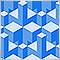Neural networks made easy (Part 37): Sparse Attention
In the previous article, we discussed relational models which use attention mechanisms in their architecture. One of the specific features of these models is the intensive utilization of computing resources. In this article, we will consider one of the mechanisms for reducing the number of computational operations inside the Self-Attention block. This will increase the general performance of the model.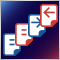Understanding order placement in MQL5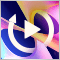Developing a Replay System — Market simulation (Part 06): First improvements (I)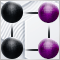Category Theory in MQL5 (Part 19): Naturality Square Induction